# R S Aggarwal Solutions for Class 11 Maths Chapter 28 Differentiation

R S Aggarwal Solutions Class 11 Chapter 28 pdf include answers to all the differentiation exercise questions. Differentiation is the process of finding the derivative. In this chapter, students will learn to find the derivative of functions using different methods. Derivatives using the first principle is one of the most important methods to find the derivative of a function, which is introduced in this chapter. This chapter is a set of five exercises where students will be seeing questions on differentiation which are covered in the pdf given below. Students are advised to download the R S Aggarwal class 11 solutions and start practicing to score good marks in the examinations.

## Download PDF of R S Aggarwal Solutions for Class 11 Maths Chapter 28 Differentiation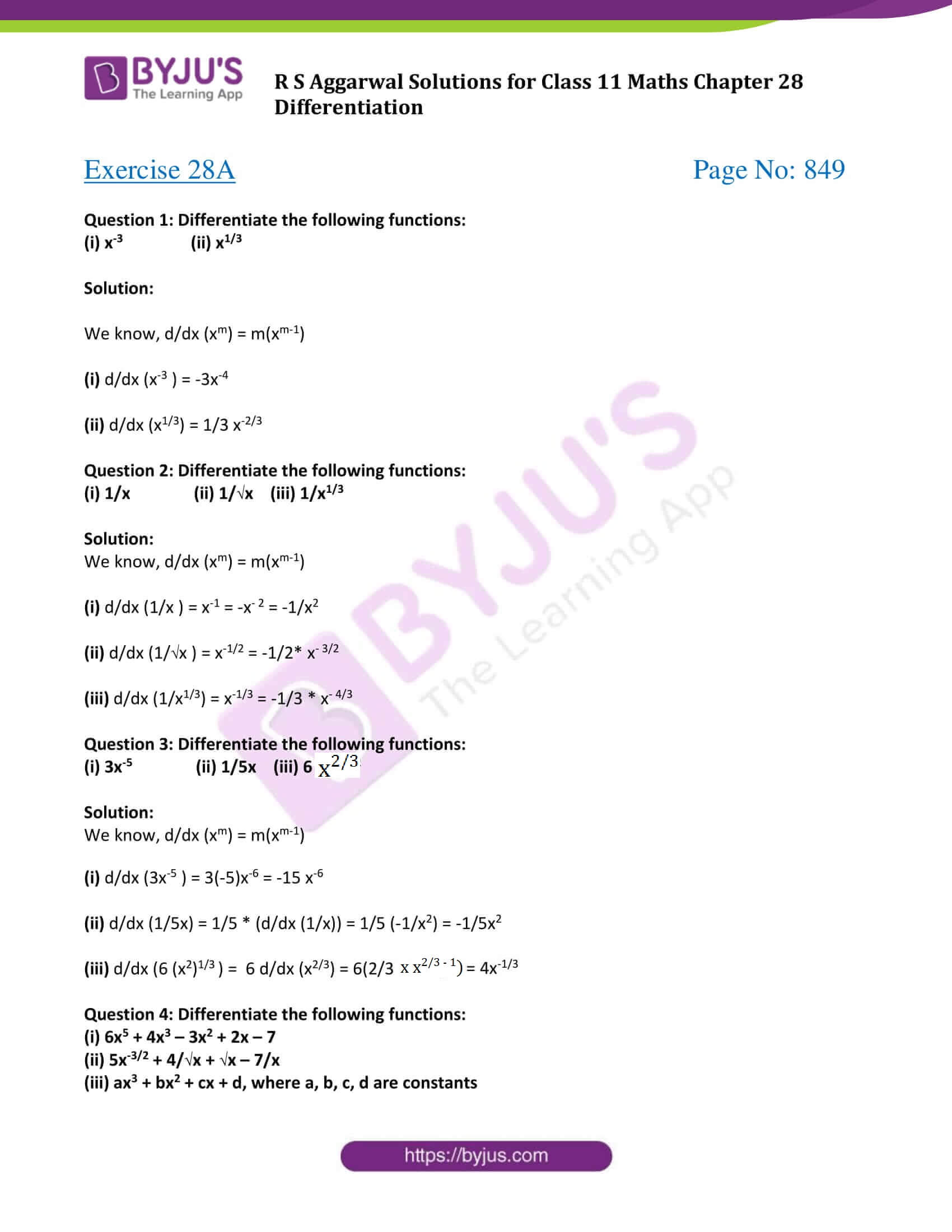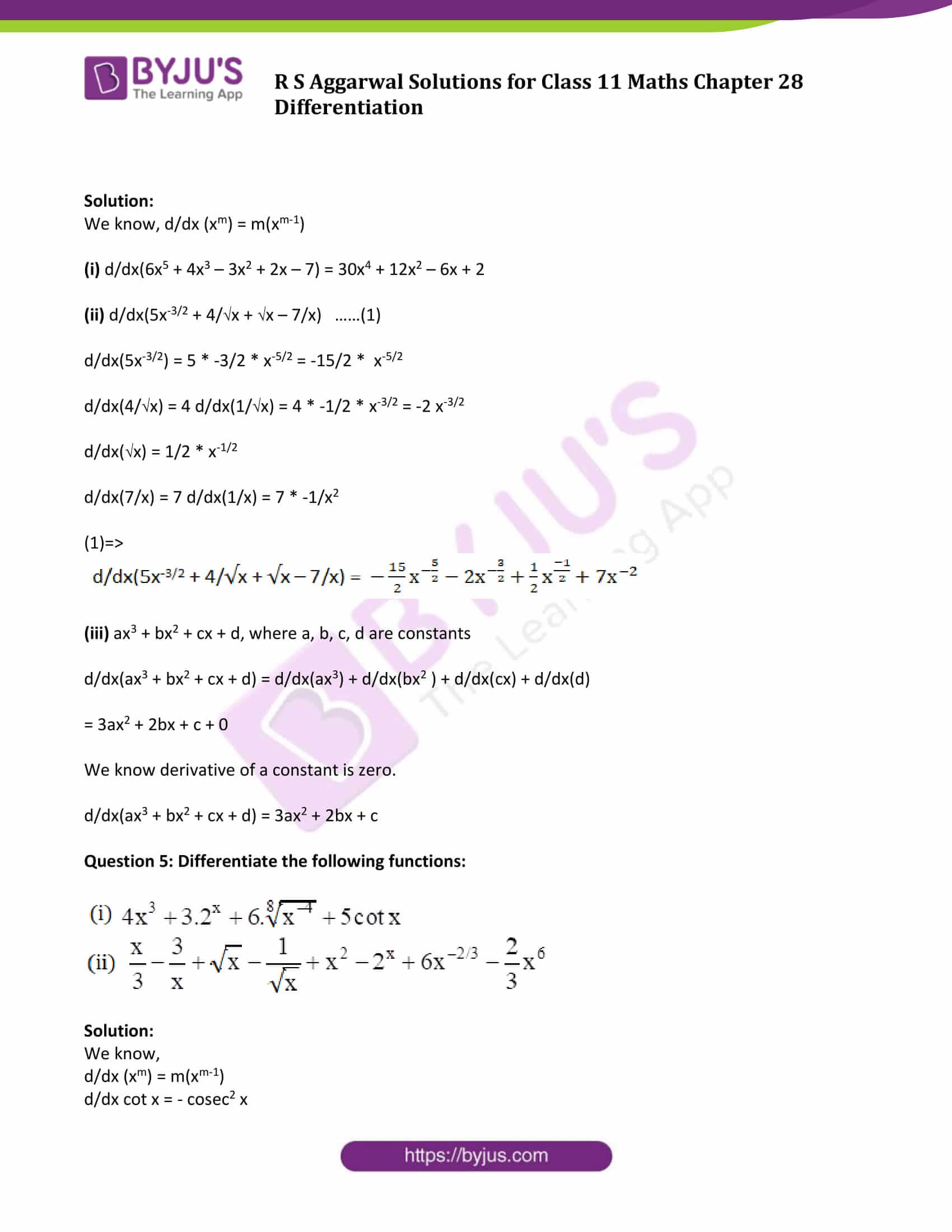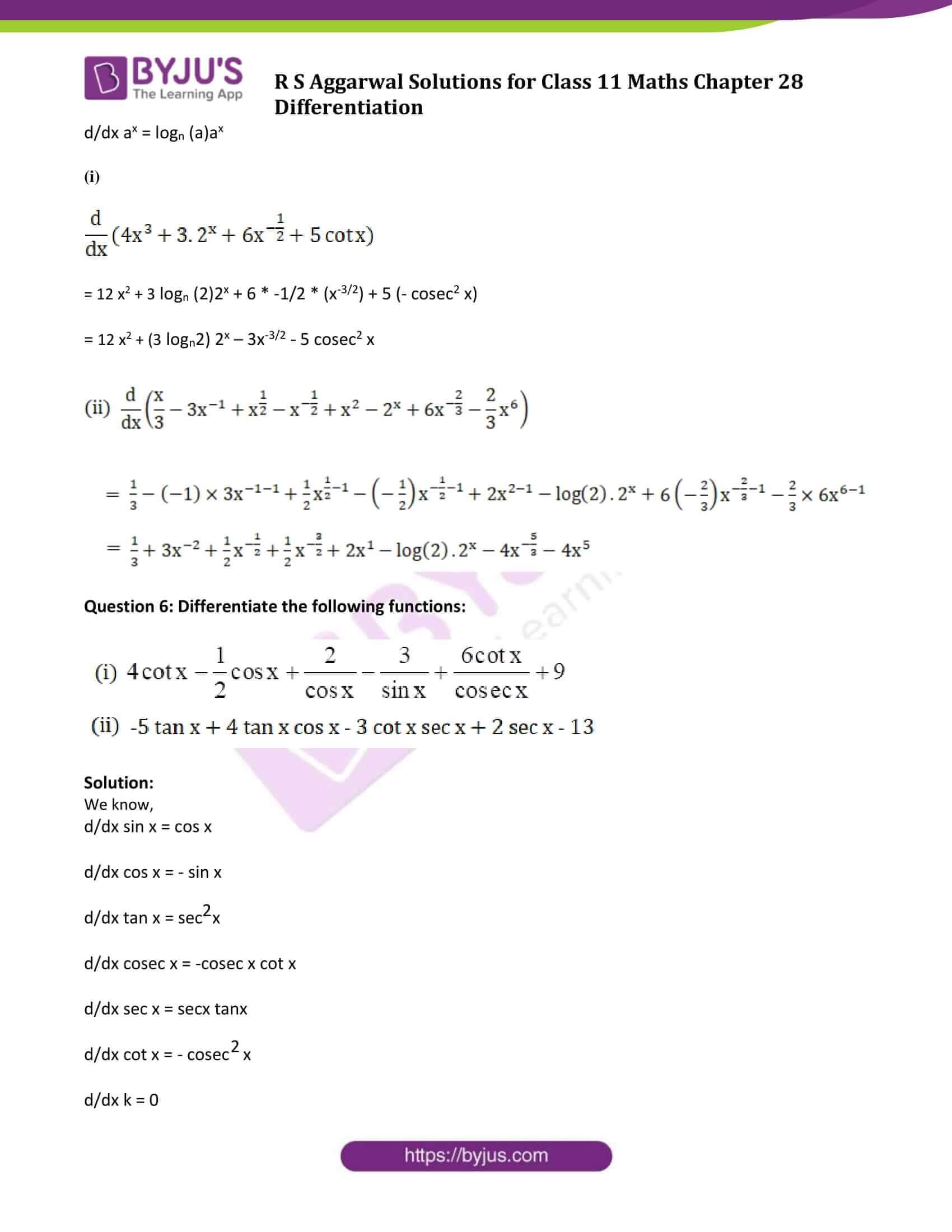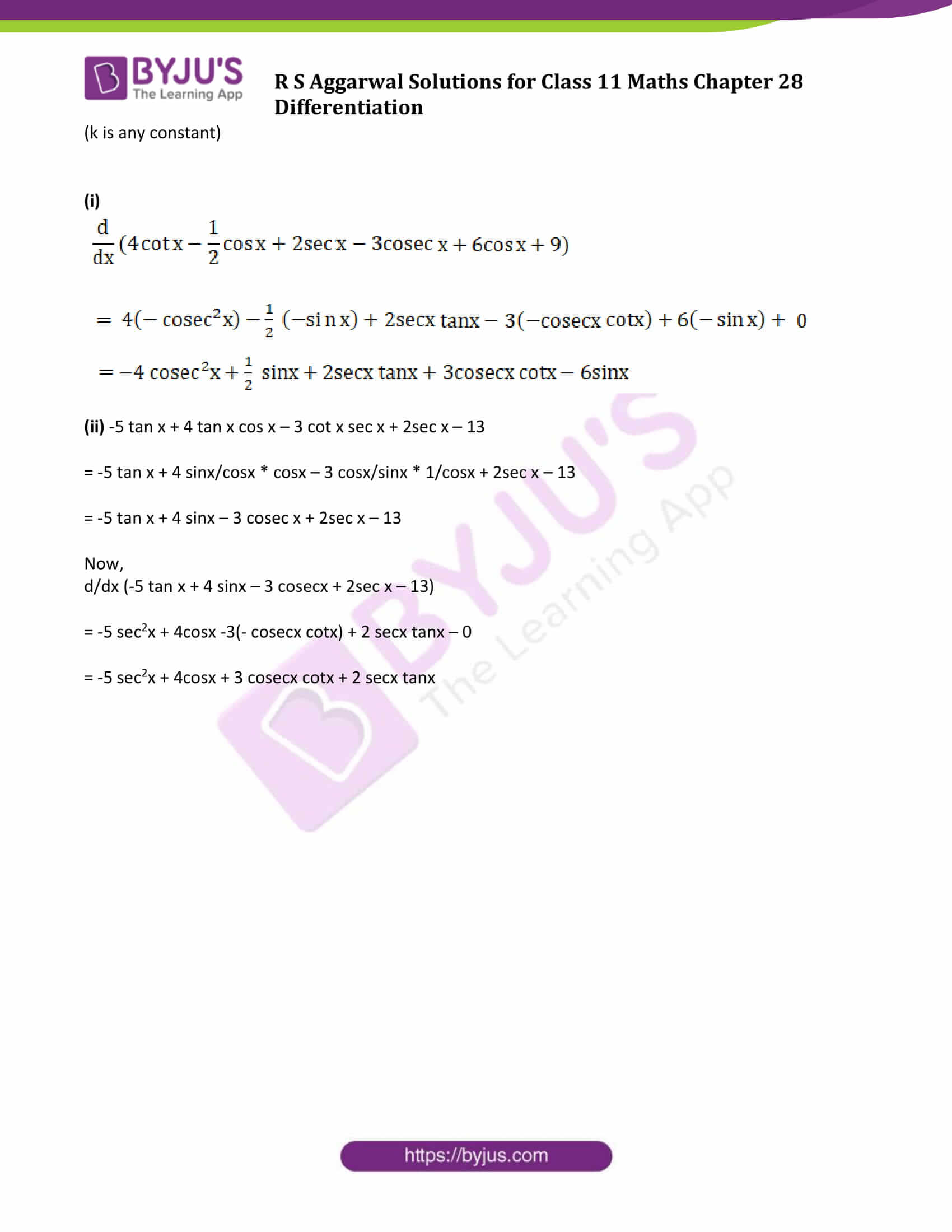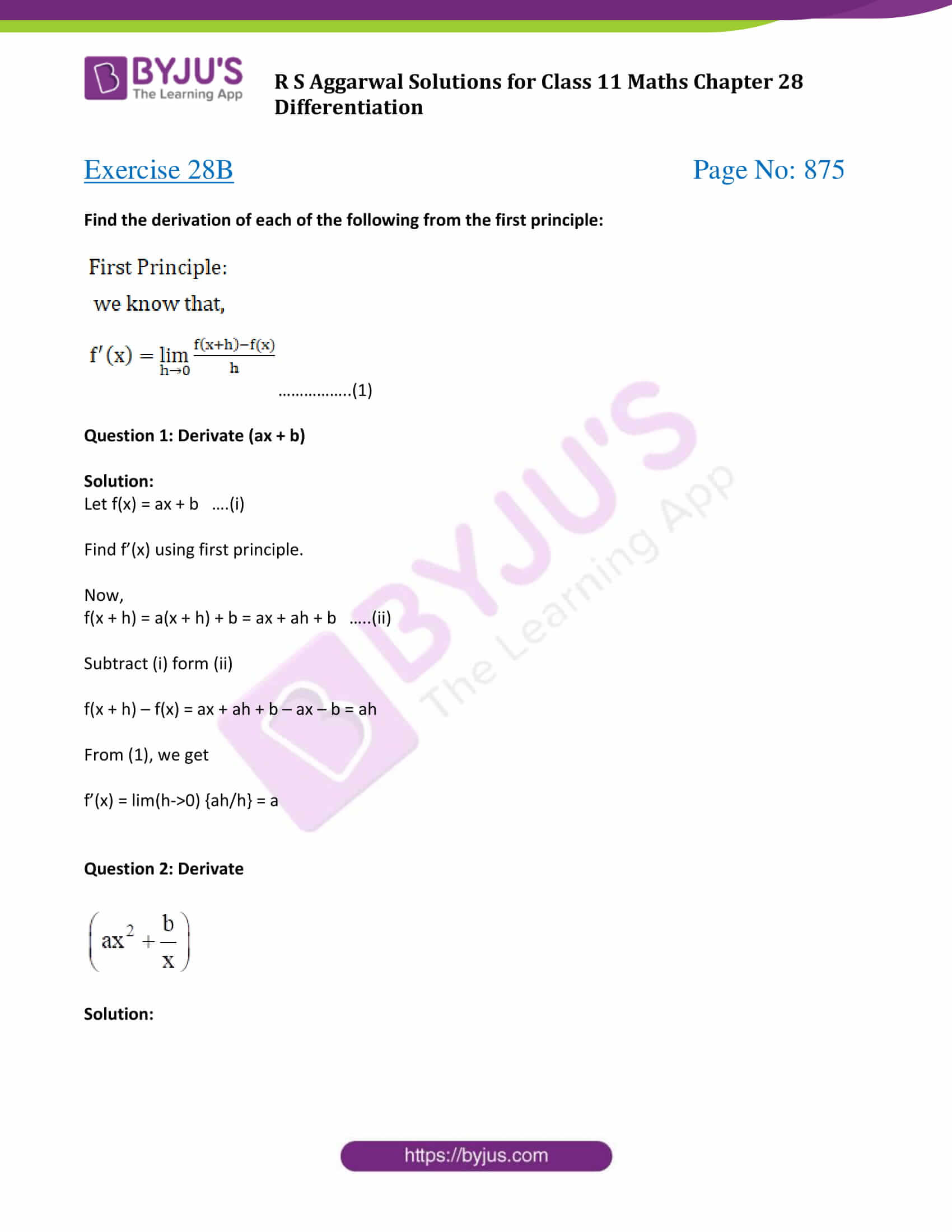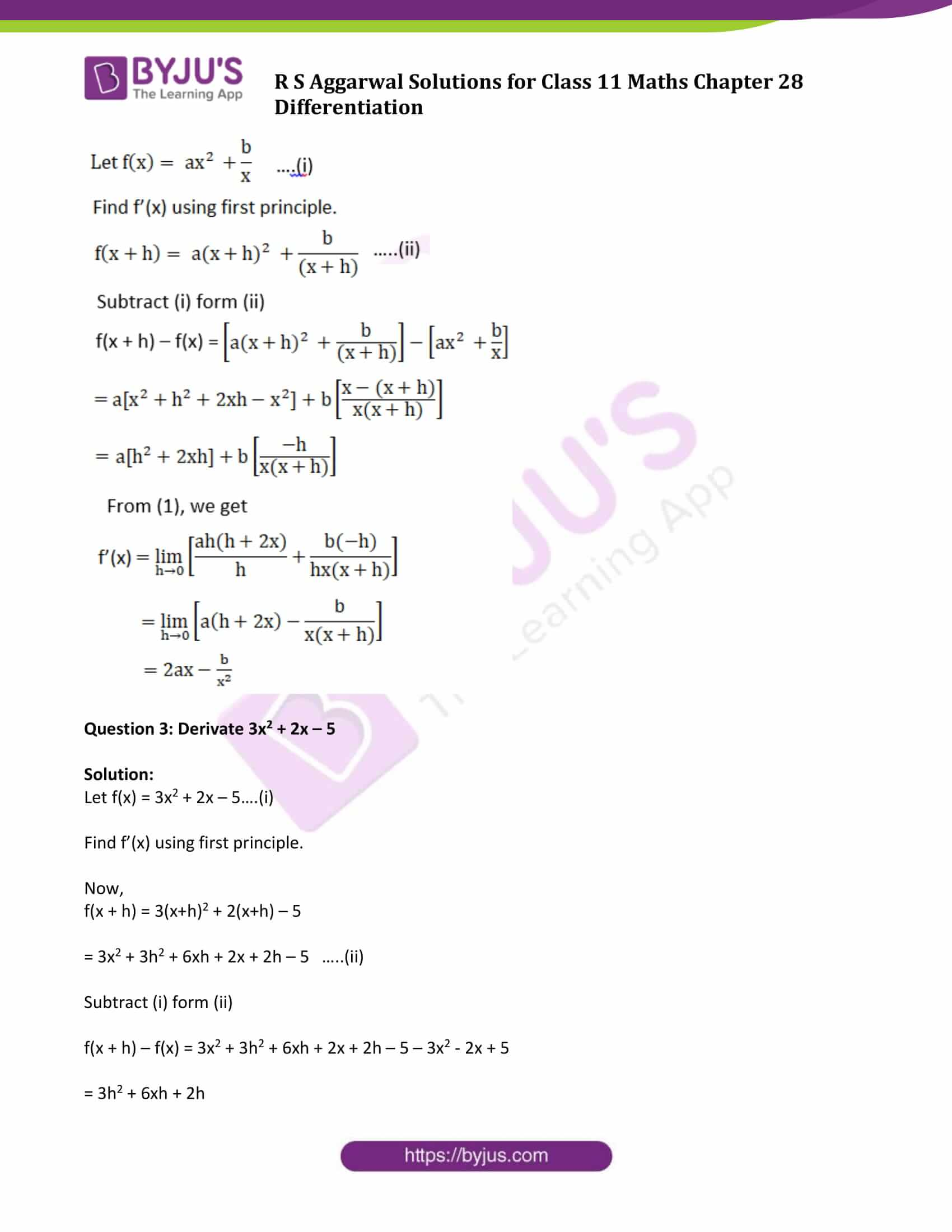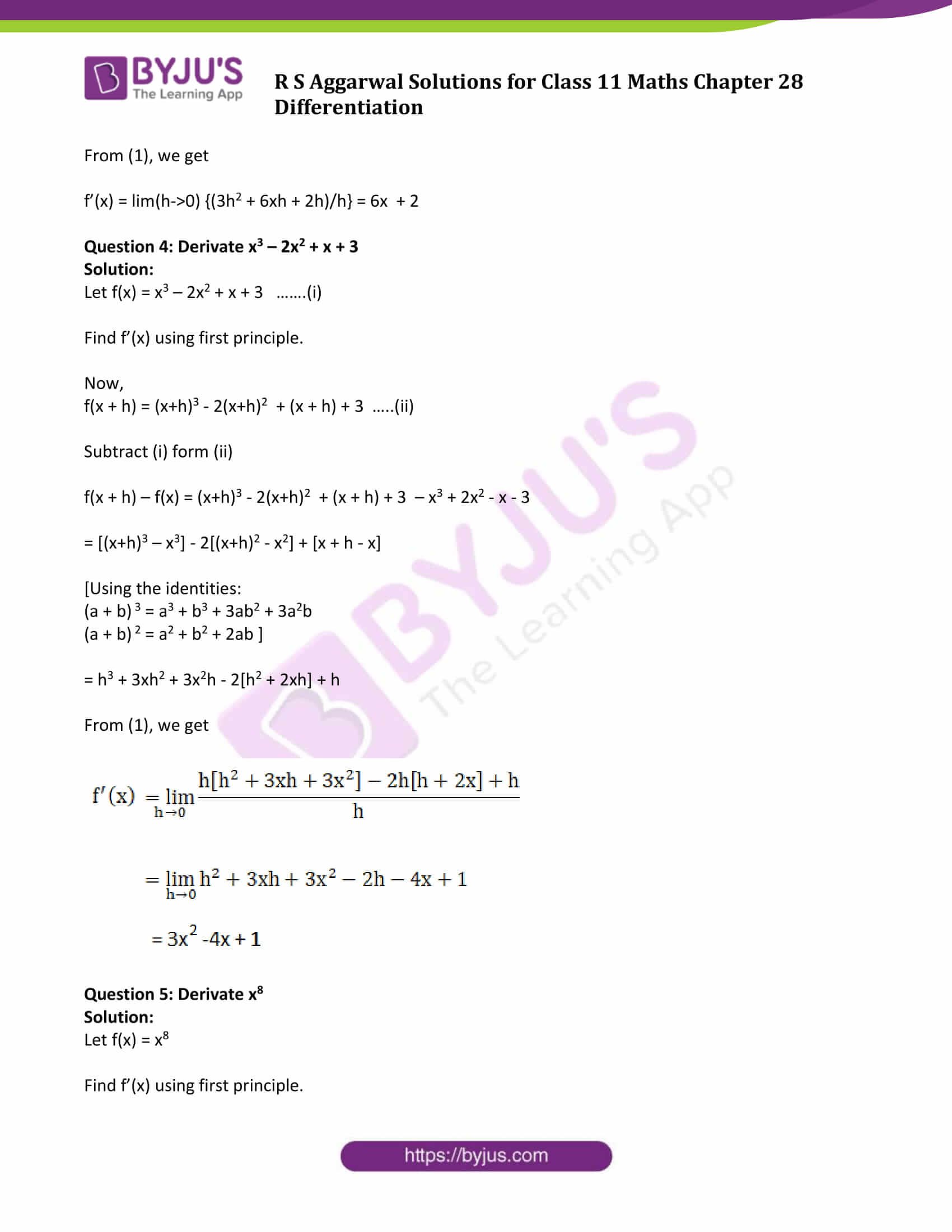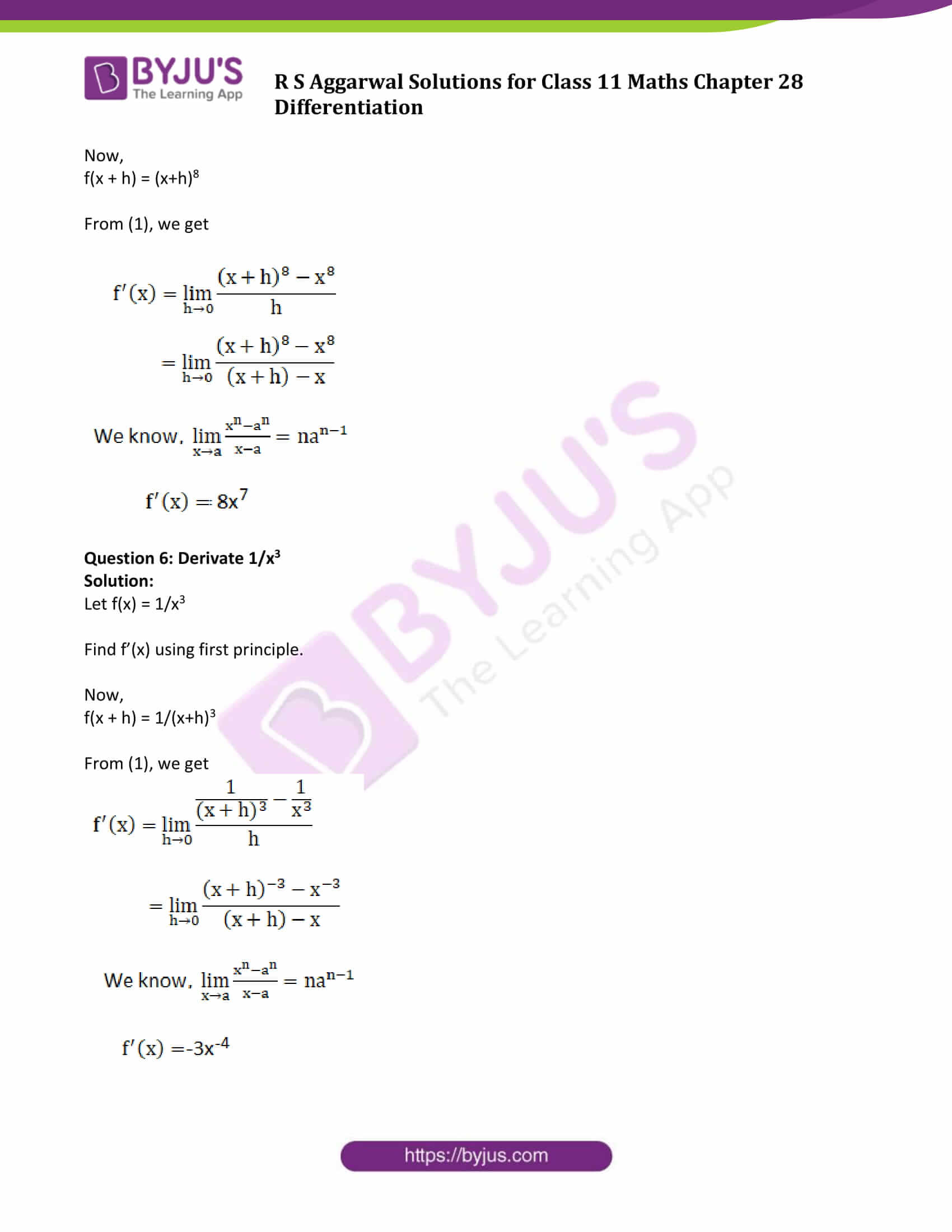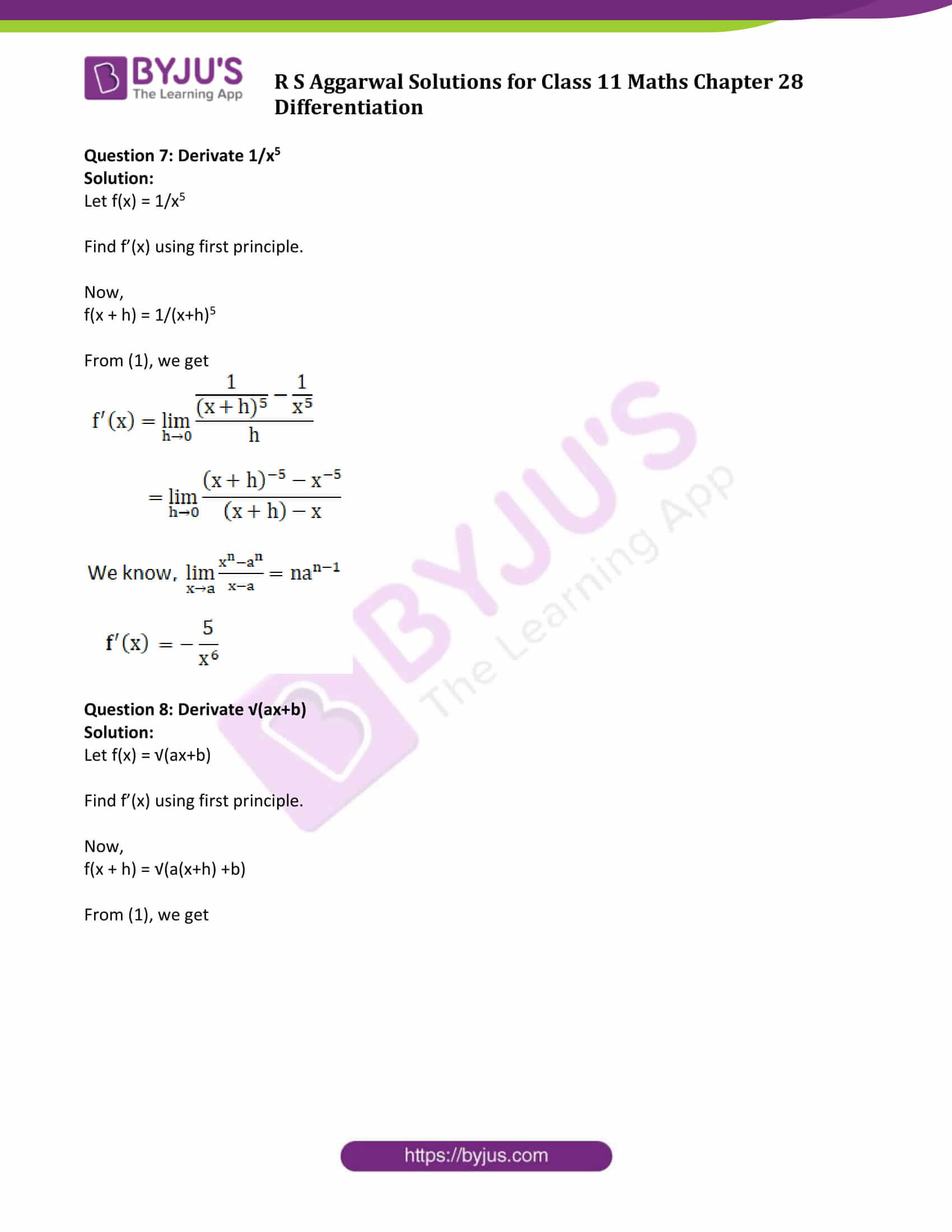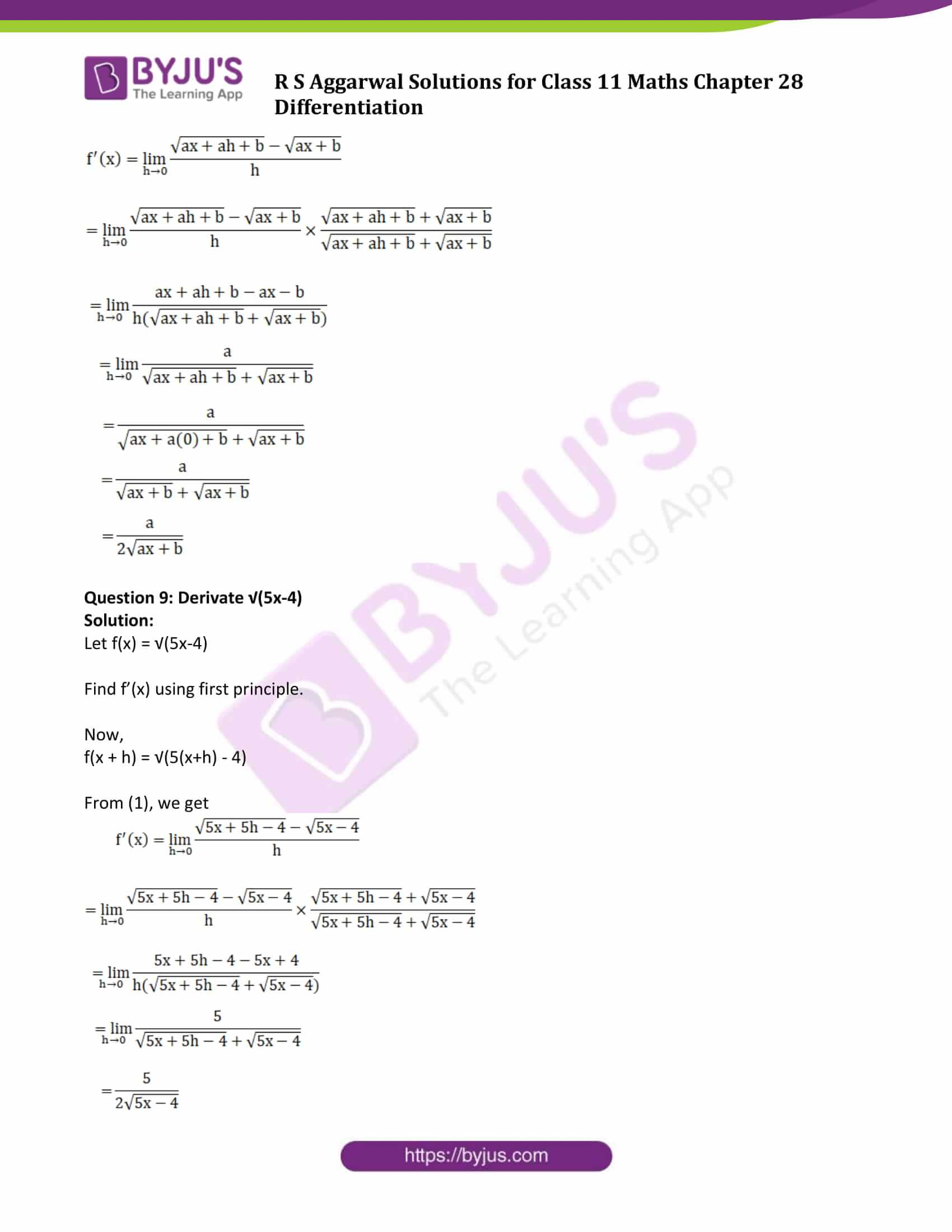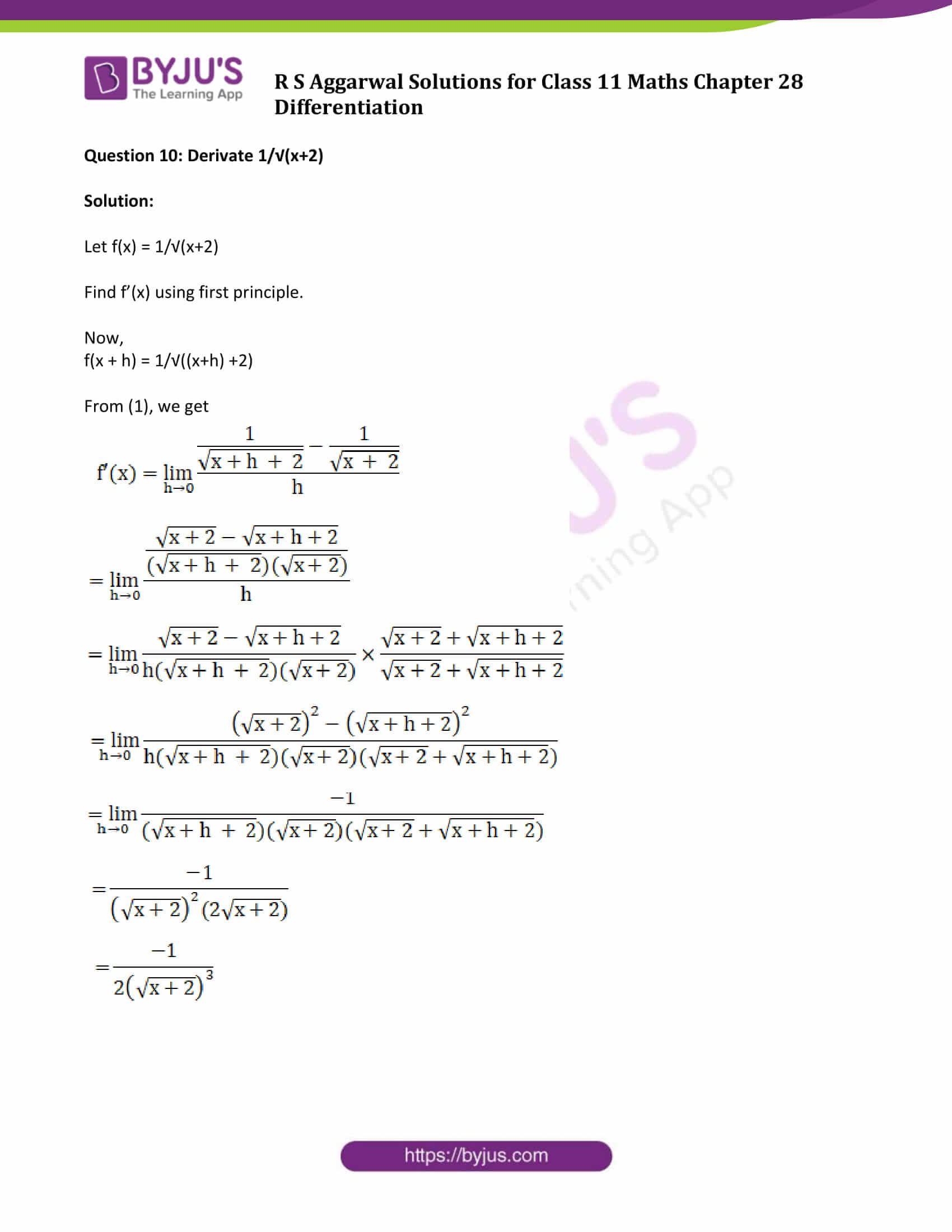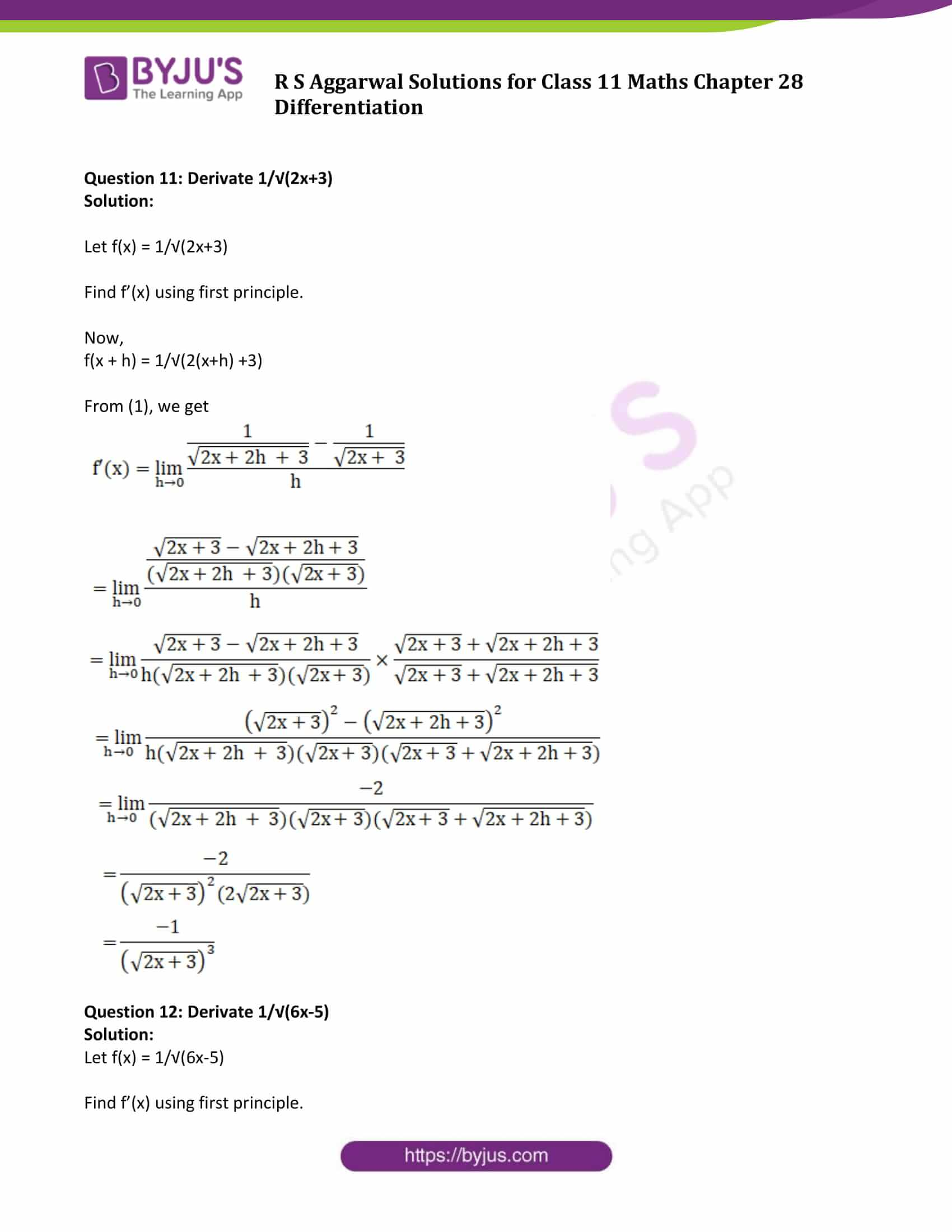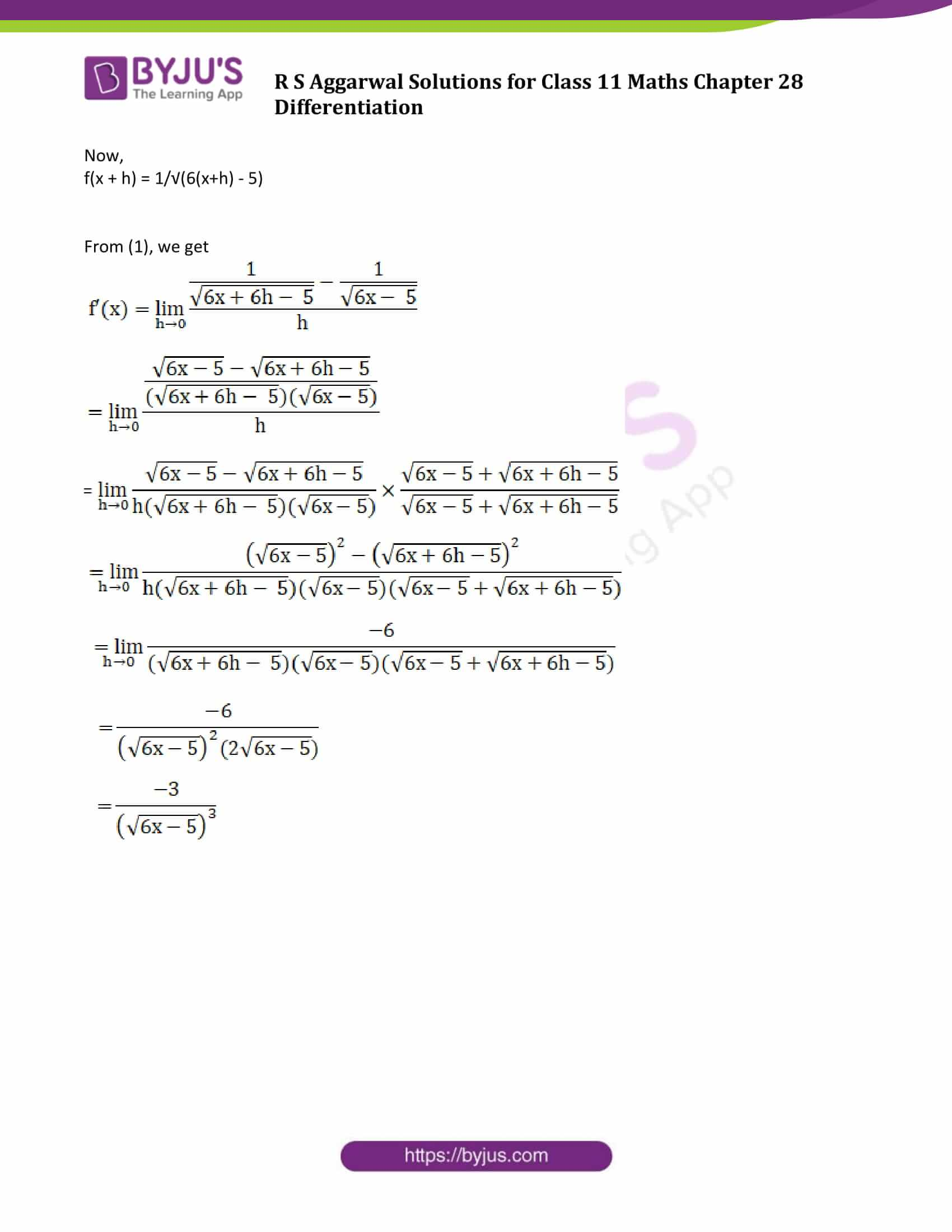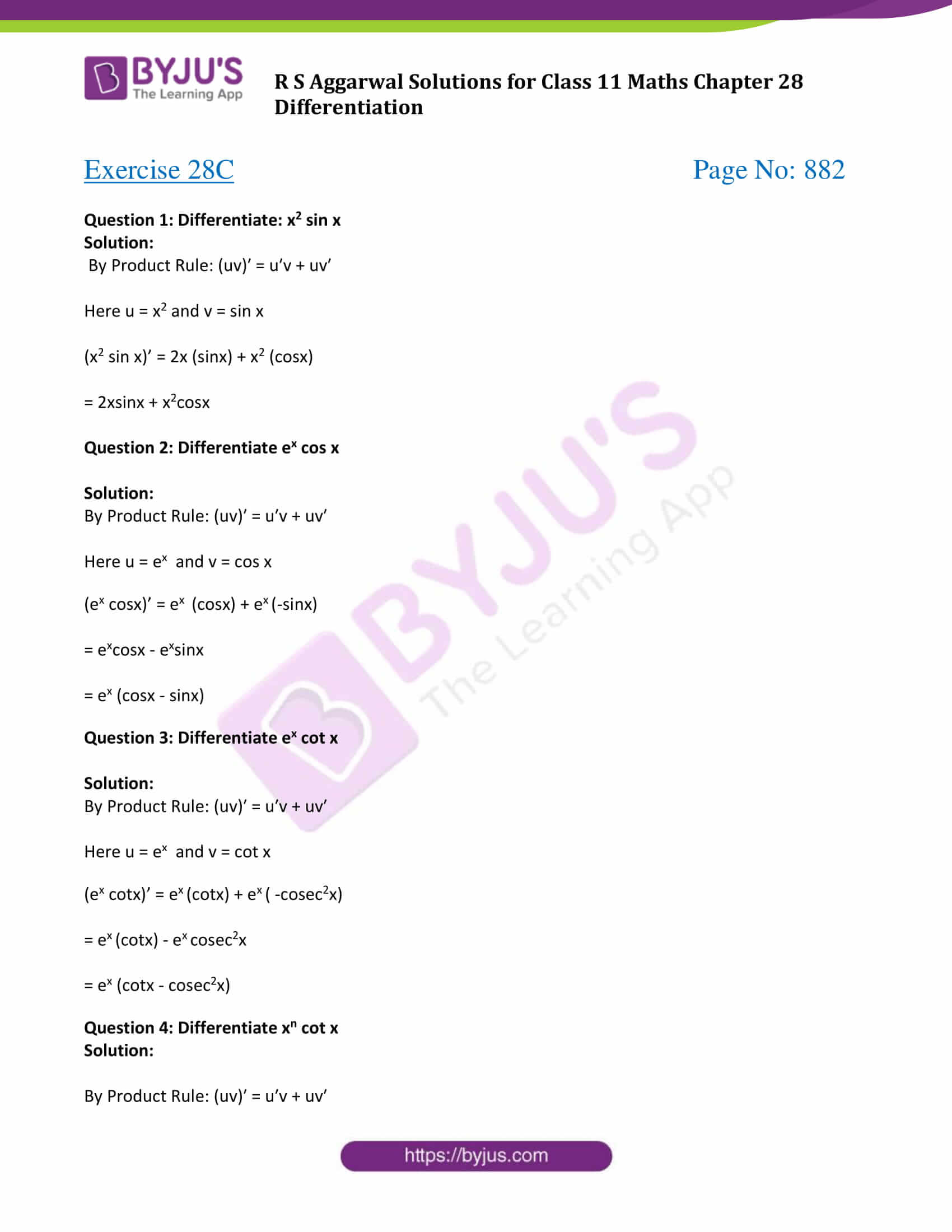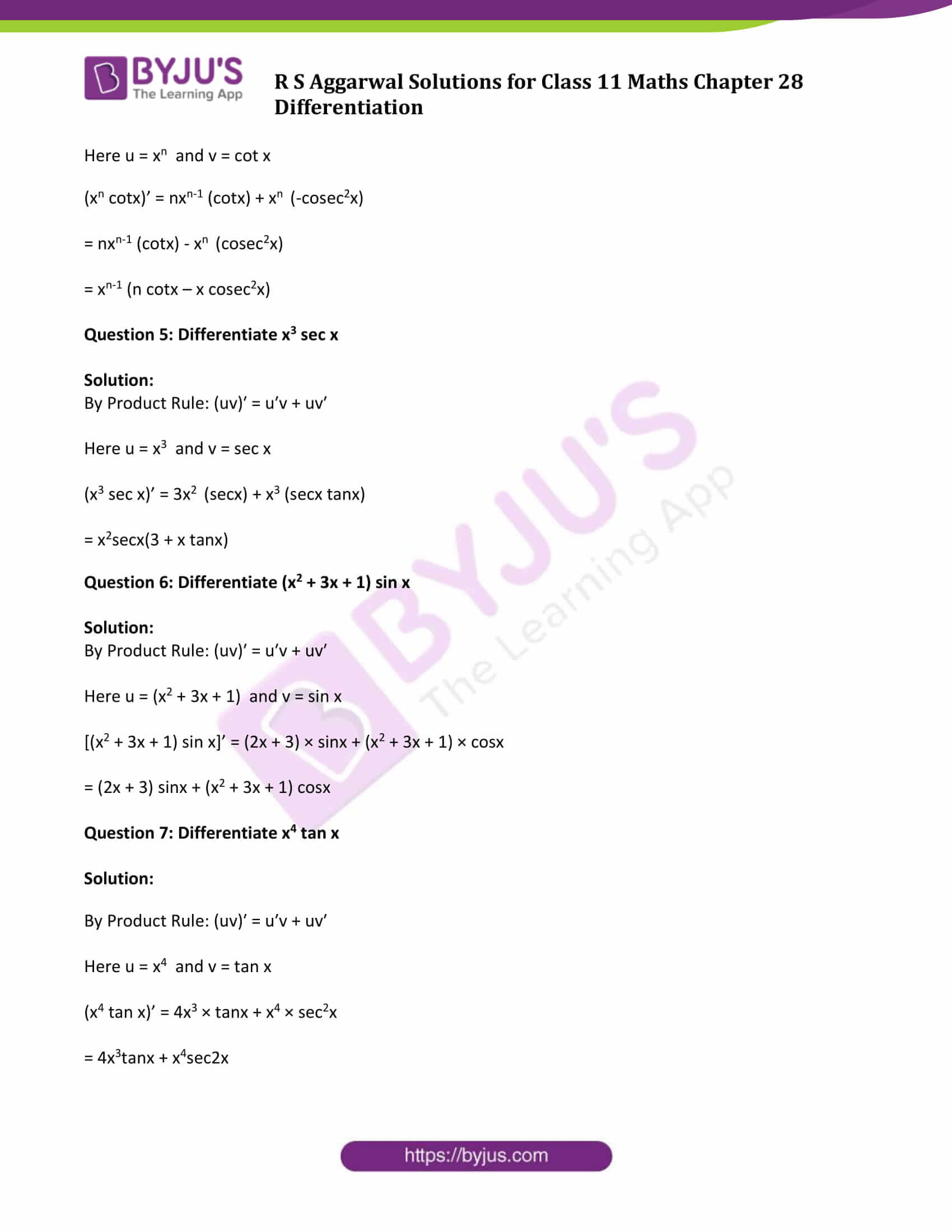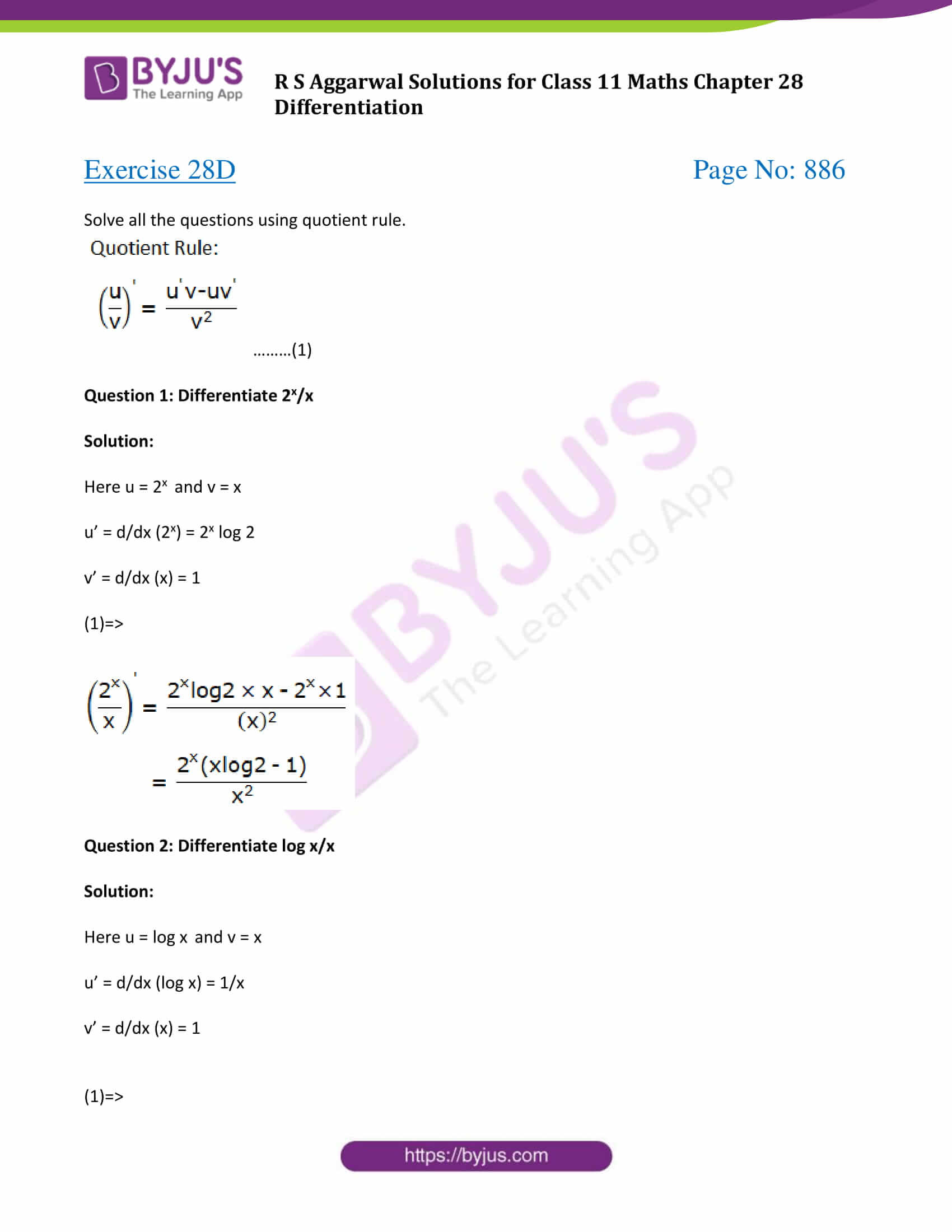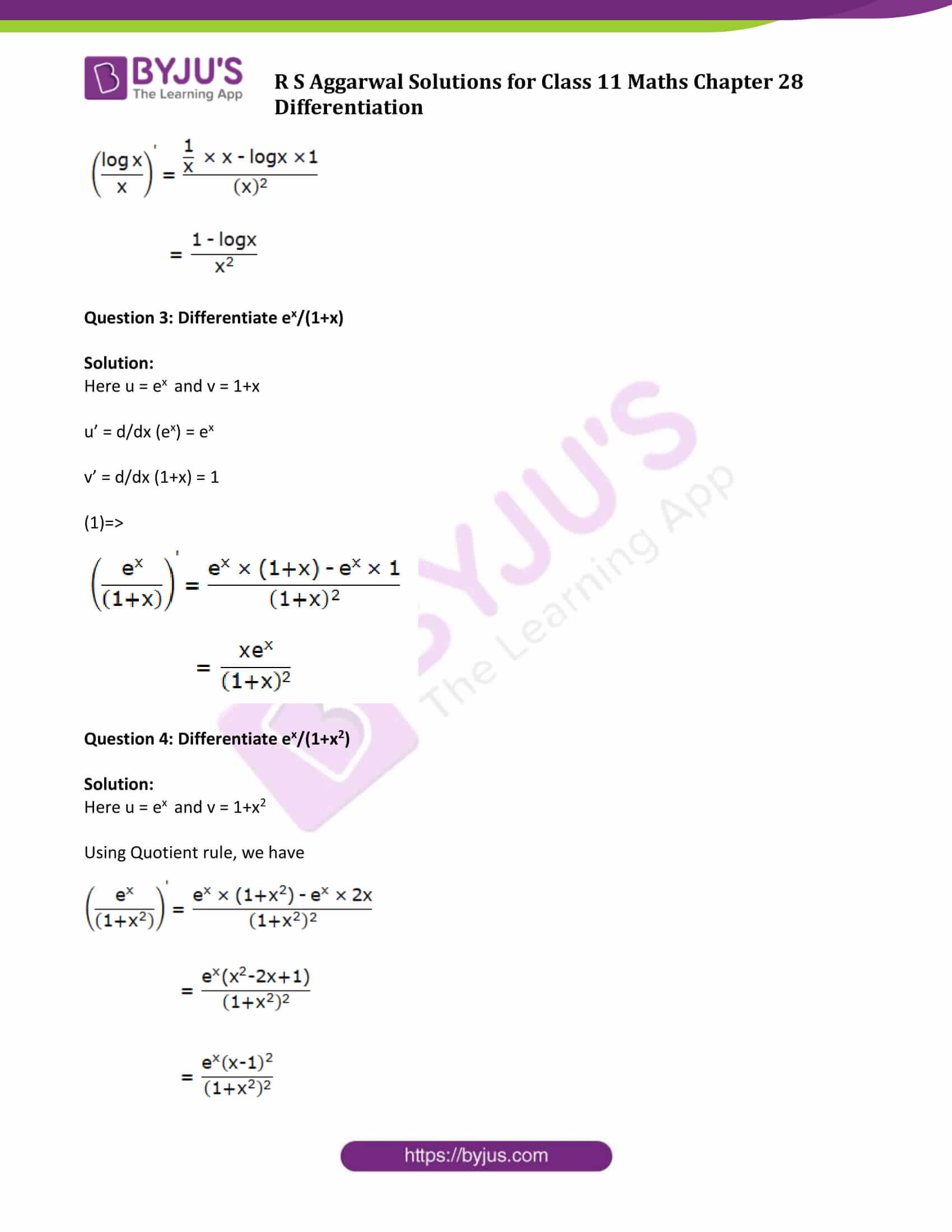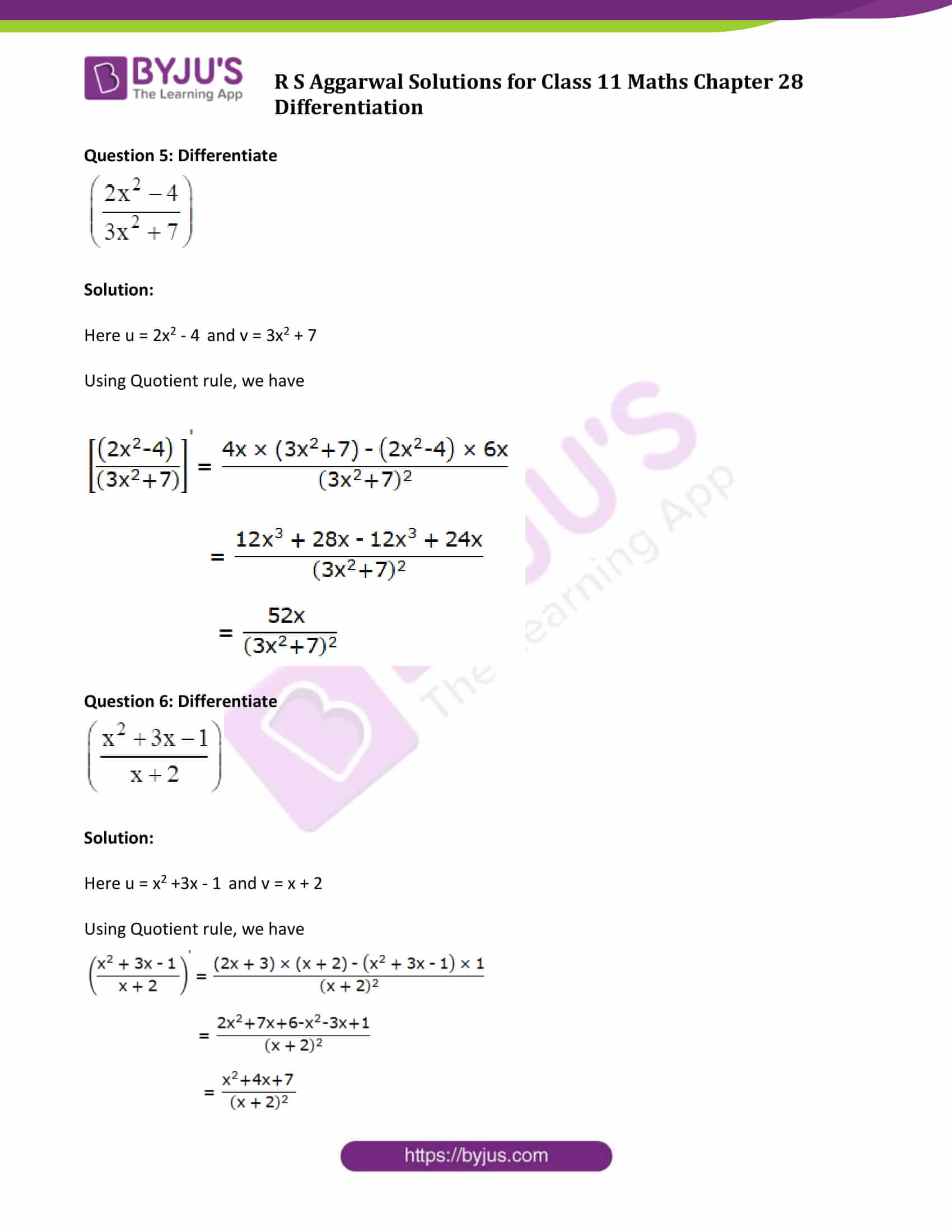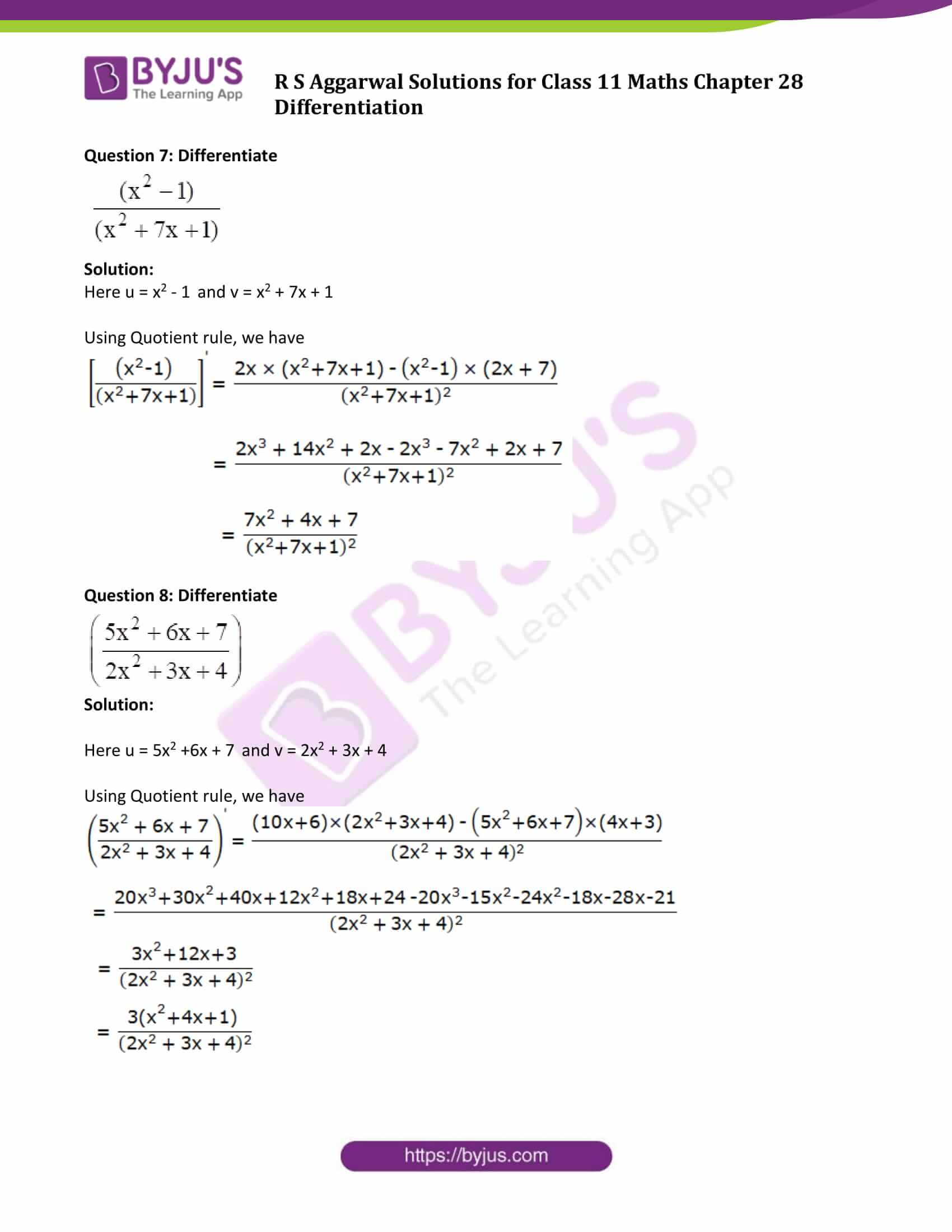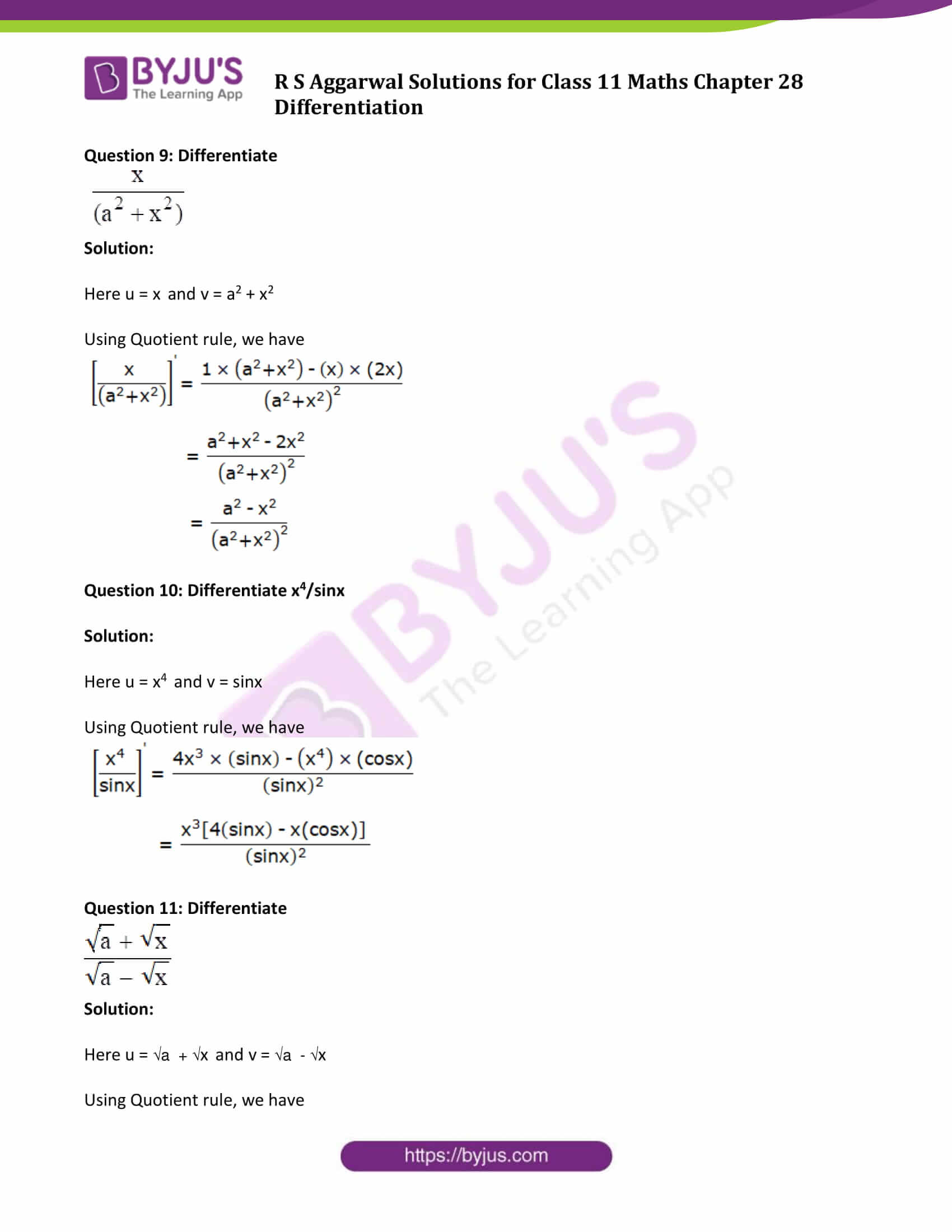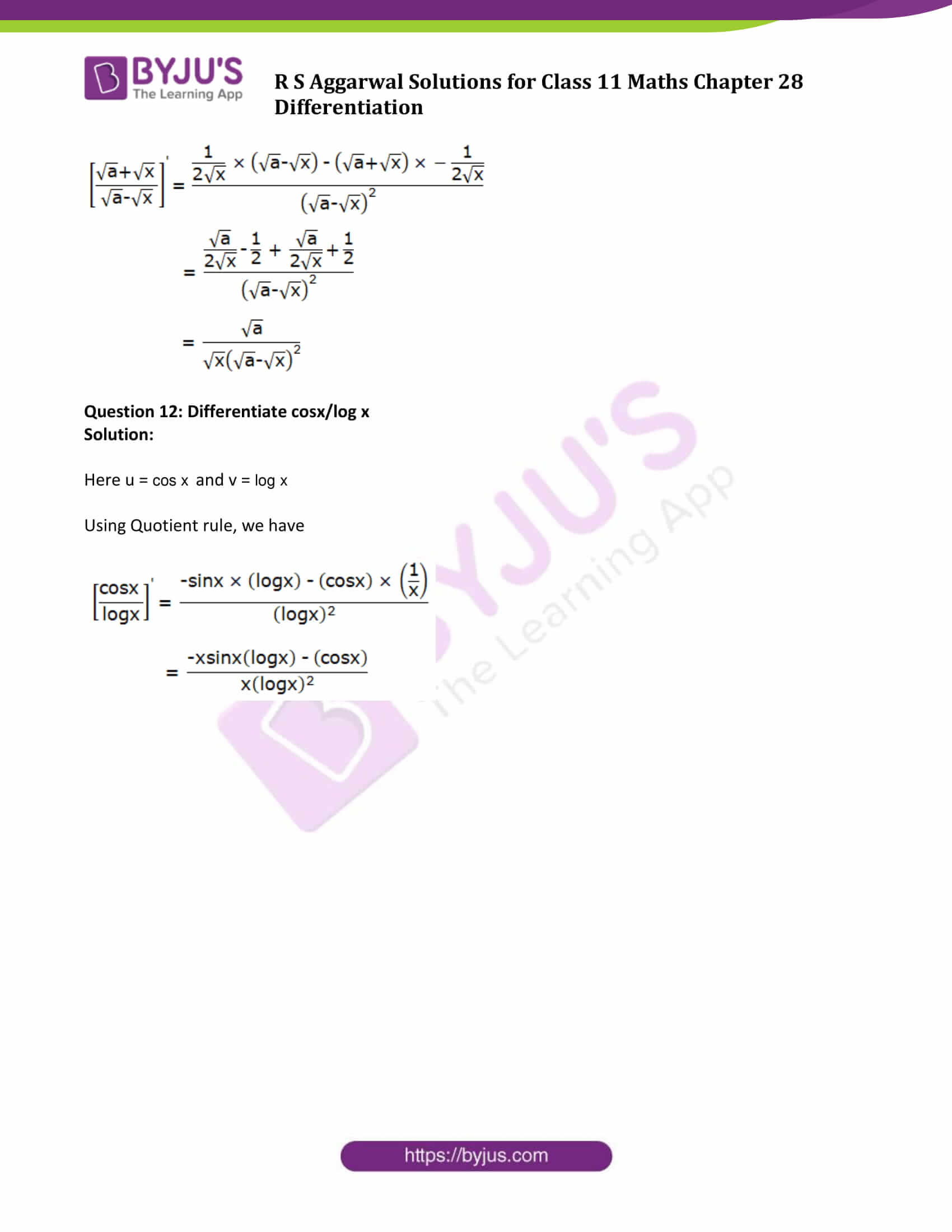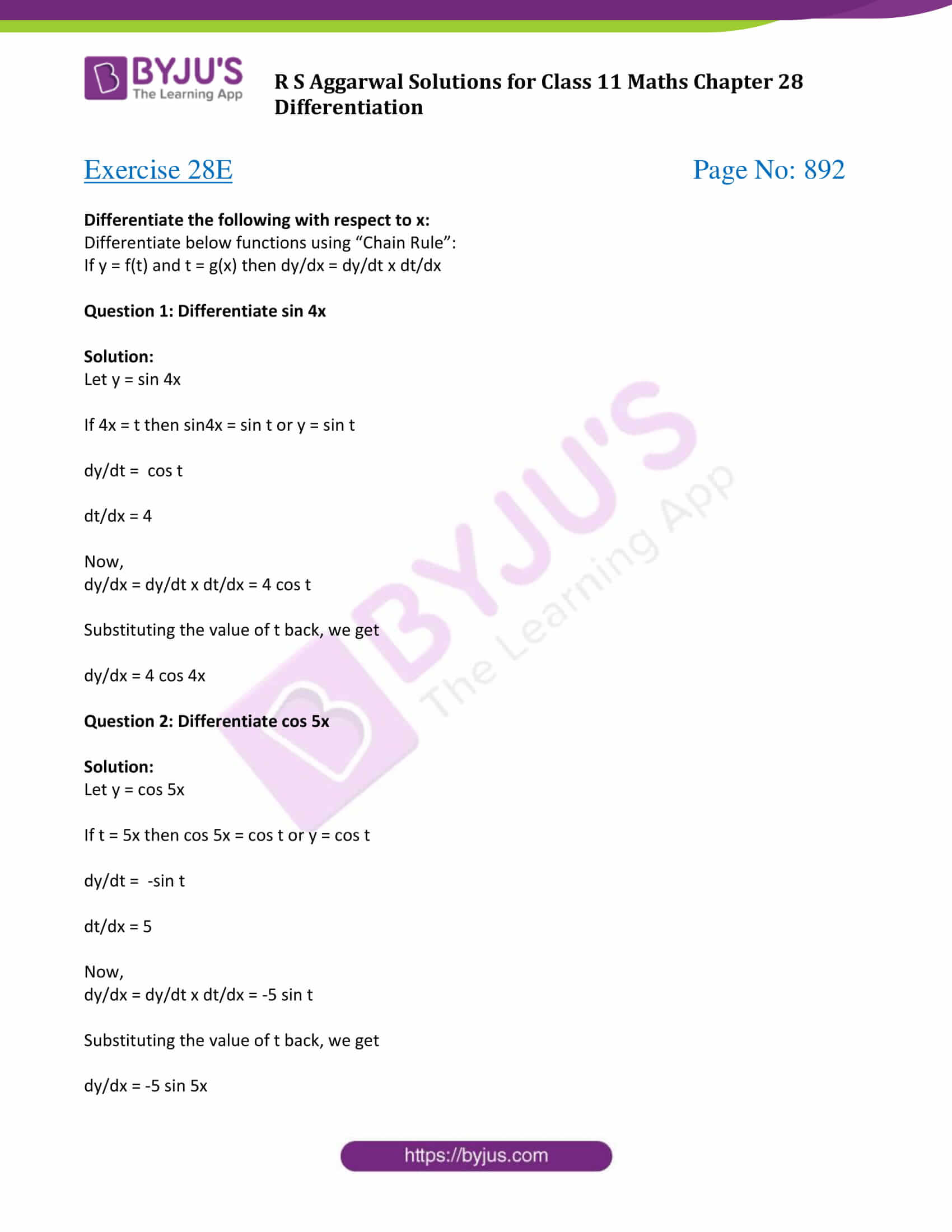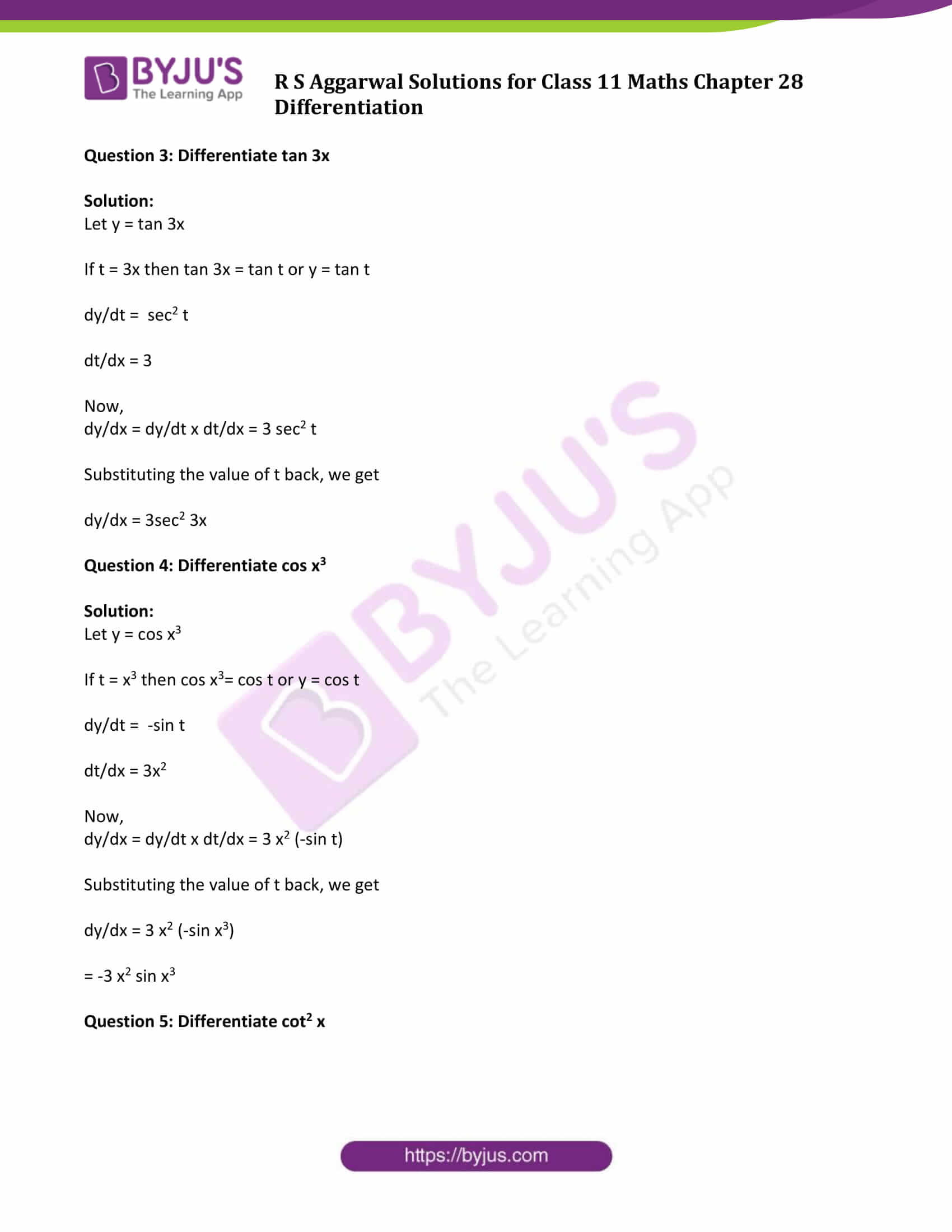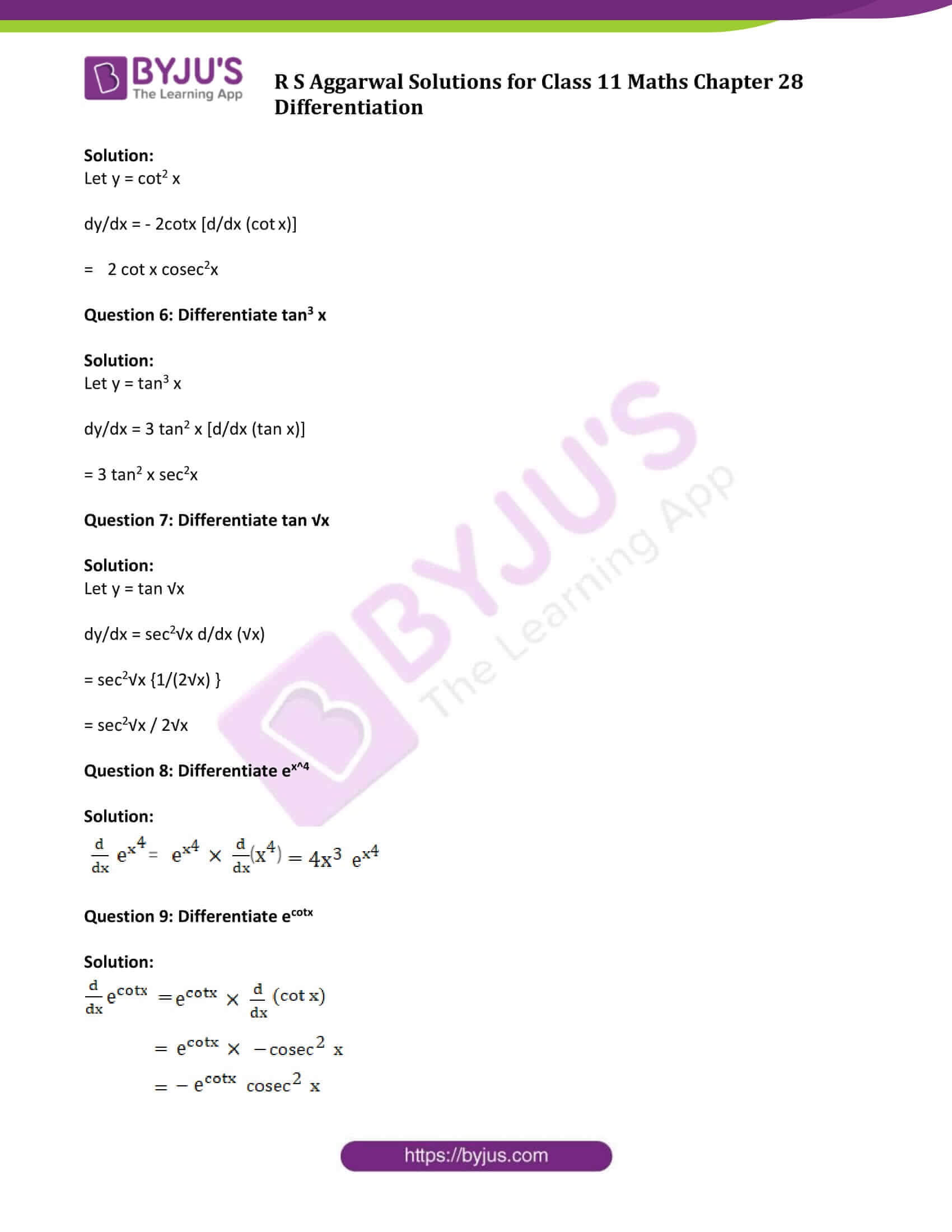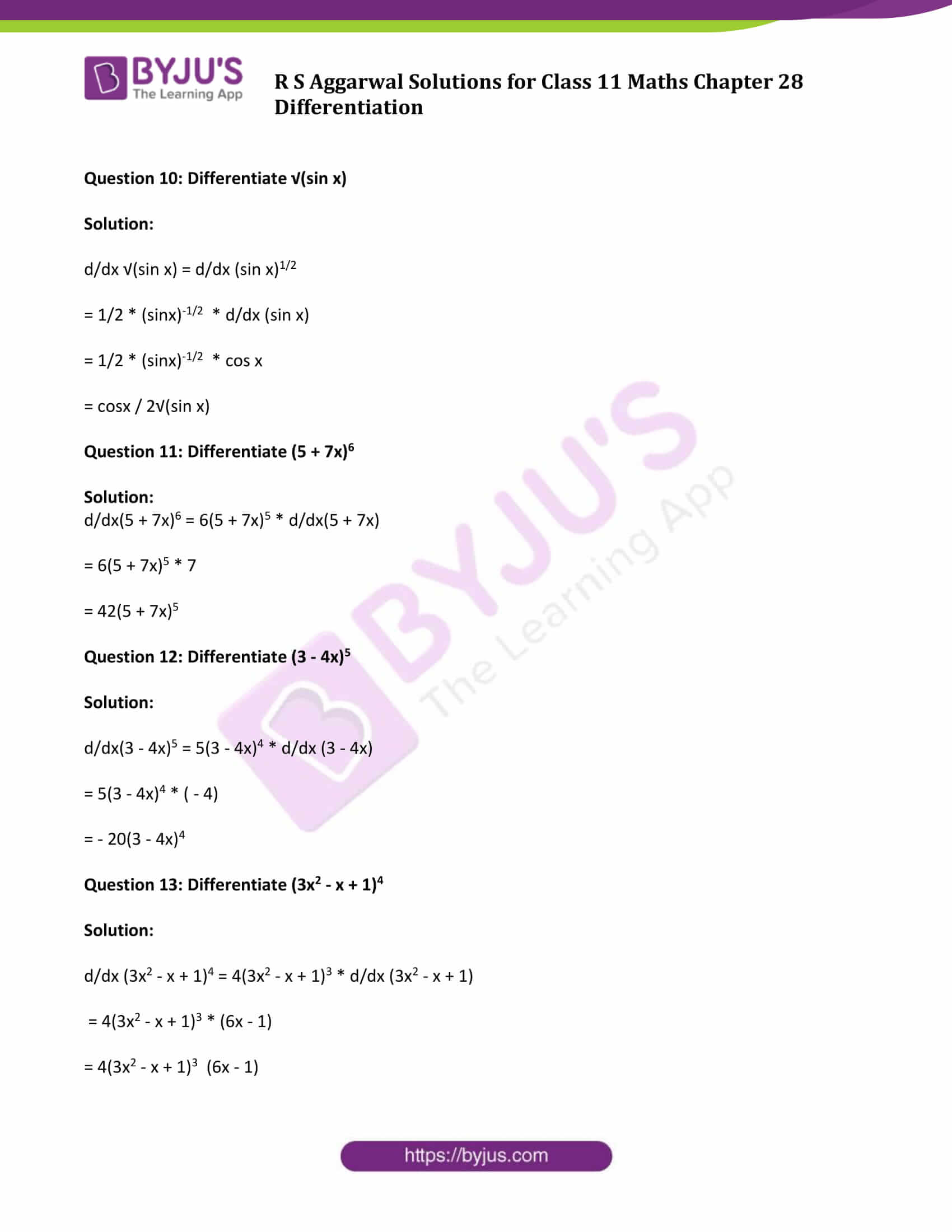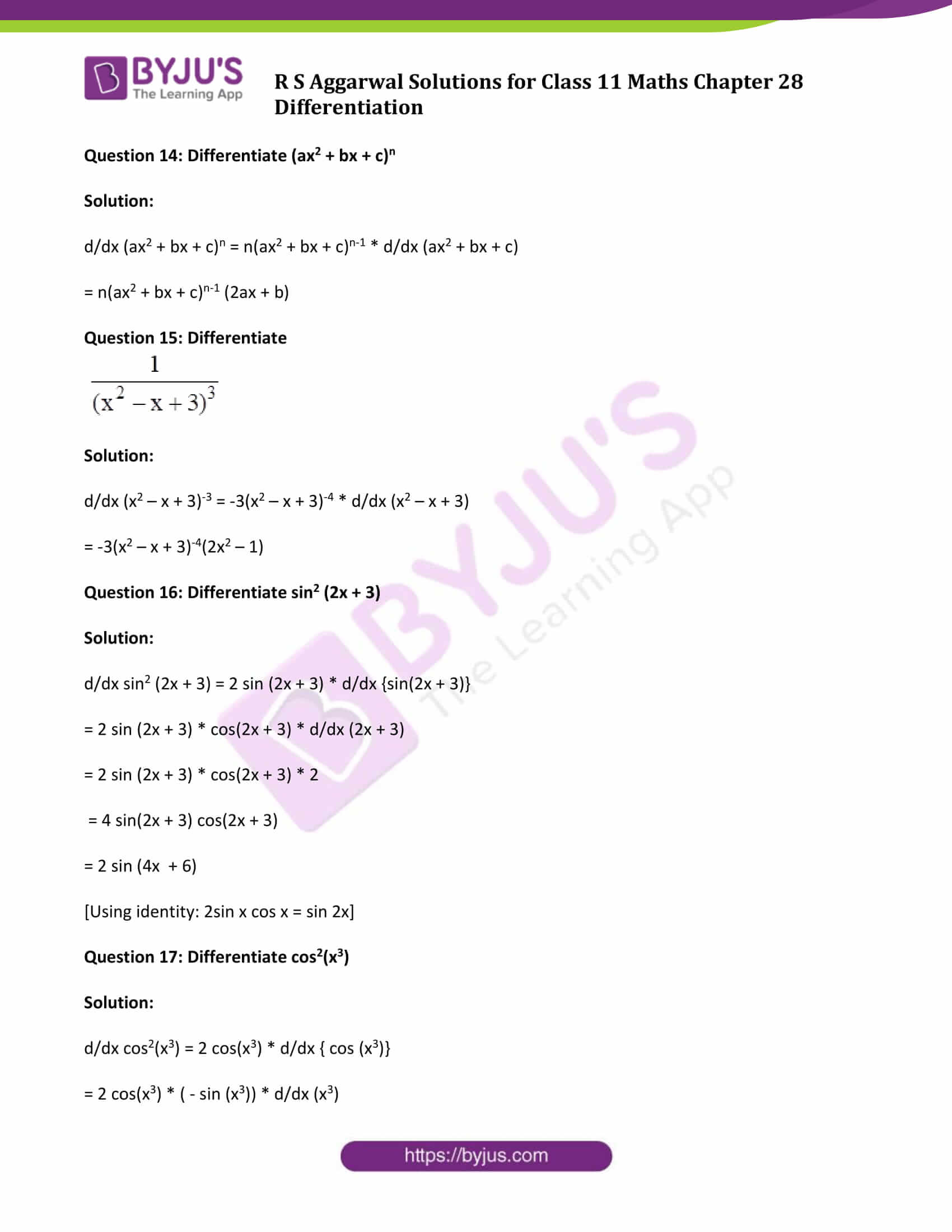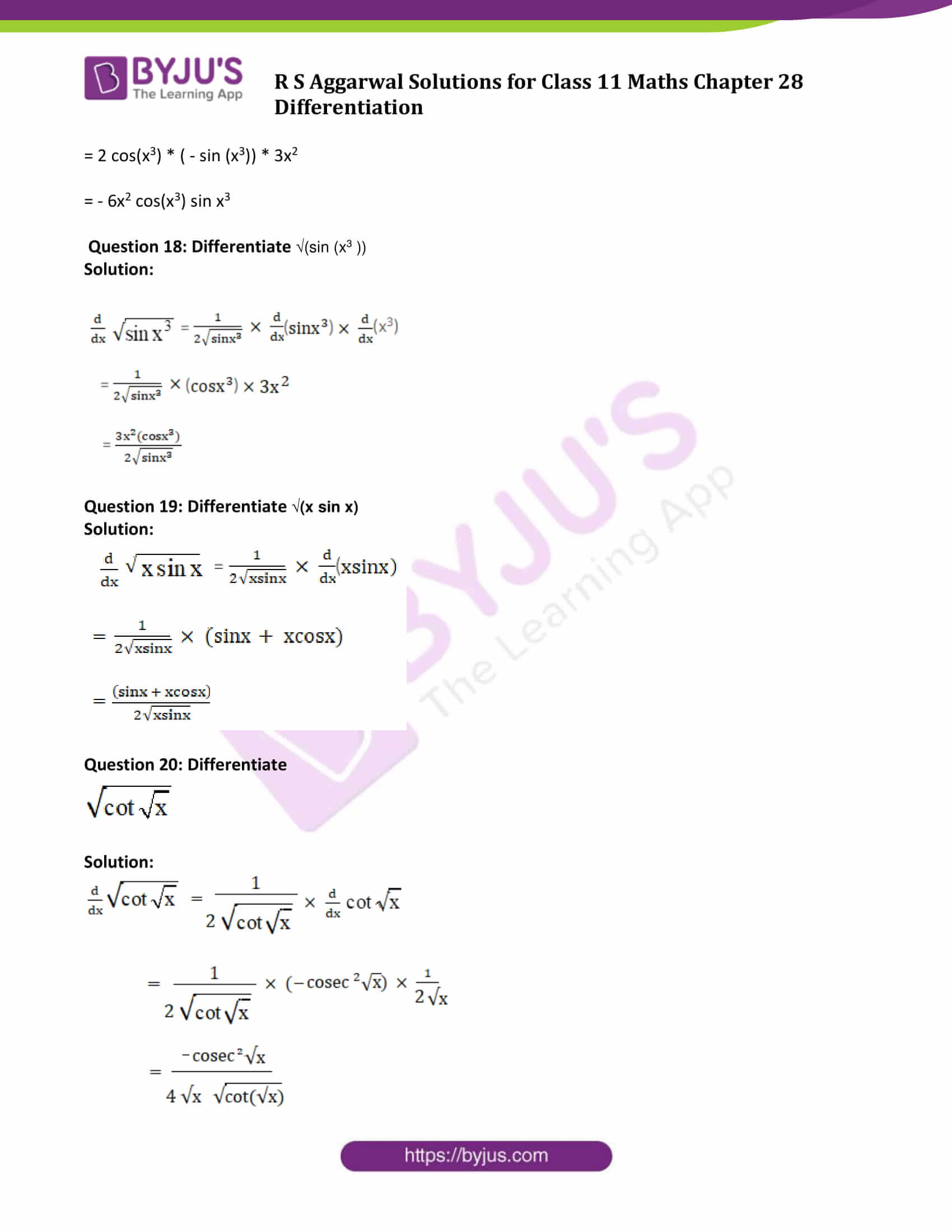### Access Answers to Maths R S Aggarwal Chapter 28 Differentiation

Exercise 28A Page No: 849

Question 1: Differentiate the following functions:

(i) x-3 (ii) x1/3

Solution:

We know, d/dx (xm) = m(xm-1)

(i) d/dx (x-3 ) = -3x-4

(ii) d/dx (x1/3) = 1/3 x-2/3

Question 2: Differentiate the following functions:

(i) 1/x (ii) 1/x (iii) 1/x1/3

Solution:

We know, d/dx (xm) = m(xm-1)

(i) d/dx (1/x ) = x-1 = -x– 2 = -1/x2

(ii) d/dx (1/√x ) = x-1/2 = -1/2* x– 3/2

(iii) d/dx (1/x1/3) = x-1/3 = -1/3 * x– 4/3

Question 3: Differentiate the following functions:

(i) 3x-5 (ii) 1/5x (iii) 6 x2/3

Solution:

We know, d/dx (xm) = m(xm-1)

(i) d/dx (3x-5 ) = 3(-5)x-6 = -15 x-6

(ii) d/dx (1/5x) = 1/5 * (d/dx (1/x)) = 1/5 (-1/x2) = -1/5x2

(iii) d/dx (6 (x2)1/3 ) = 6 d/dx (x2/3) = 6(2/3 x x2/3-1) = 4x-1/3

Question 4: Differentiate the following functions:

(i) 6x5 + 4x3 – 3x2 + 2x – 7

(ii) 5x-3/2 + 4/√x + √x – 7/x

(iii) ax3 + bx2 + cx + d, where a, b, c, d are constants

Solution:

We know, d/dx (xm) = m(xm-1)

(i) d/dx(6x5 + 4x3 – 3x2 + 2x – 7) = 30x4 + 12x2 – 6x + 2

(ii) d/dx(5x-3/2 + 4/√x + √x – 7/x) ……(1)

d/dx(5x-3/2) = 5 * -3/2 * x-5/2 = -15/2 * x-5/2

d/dx(4/√x) = 4 d/dx(1/√x) = 4 * -1/2 * x-3/2 = -2 x-3/2

d/dx(√x) = 1/2 * x-1/2

d/dx(7/x) = 7 d/dx(1/x) = 7 * -1/x2

(1)=>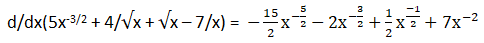(iii) ax3 + bx2 + cx + d, where a, b, c, d are constants

d/dx(ax3 + bx2 + cx + d) = d/dx(ax3) + d/dx(bx2 ) + d/dx(cx) + d/dx(d)

= 3ax2 + 2bx + c + 0

We know derivative of a constant is zero.

d/dx(ax3 + bx2 + cx + d) = 3ax2 + 2bx + c

Question 5: Differentiate the following functions: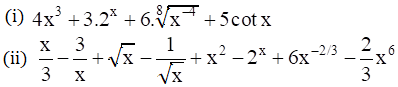Solution:

We know,

d/dx (xm) = m(xm-1)

d/dx cot x = – cosec2 x

d/dx ax = logn (a)ax

(i)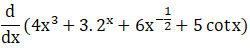= 12 x2 + 3 logn (2)2x + 6 * -1/2 * (x-3/2) + 5 (- cosec2 x)

= 12 x2 + (3 logn2) 2x – 3x-3/2 – 5 cosec2 x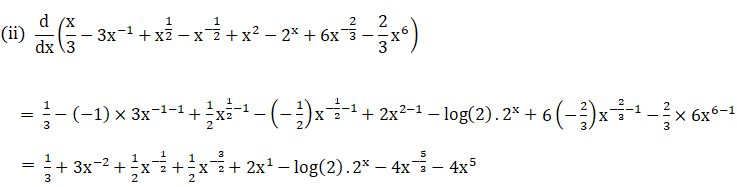Question 6: Differentiate the following functions: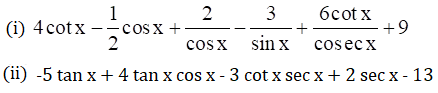Solution:

We know,

d/dx sin x = cos x

d/dx cos x = – sin x

d/dx tan x = sec2x

d/dx cosec x = -cosec x cot x

d/dx sec x = secx tanx

d/dx cot x = – cosec2 x

d/dx k = 0

(k is any constant)

(i)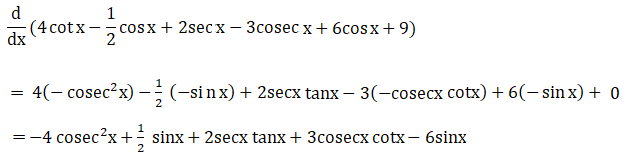(ii) -5 tan x + 4 tan x cos x – 3 cot x sec x + 2sec x – 13

= -5 tan x + 4 sinx/cosx * cosx – 3 cosx/sinx * 1/cosx + 2sec x – 13

= -5 tan x + 4 sinx – 3 cosec x + 2sec x – 13

Now,

d/dx (-5 tan x + 4 sinx – 3 cosecx + 2sec x – 13)

= -5 sec2x + 4cosx -3(- cosecx cotx) + 2 secx tanx – 0

= -5 sec2x + 4cosx + 3 cosecx cotx + 2 secx tanx

Exercise 28B Page No: 875

Find the derivation of each of the following from the first principle: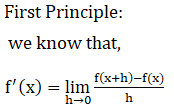……………..(1)

Question 1: Derivate (ax + b)

Solution:

Let f(x) = ax + b ….(i)

Find f’(x) using first principle.

Now,

f(x + h) = a(x + h) + b = ax + ah + b …..(ii)

Subtract (i) form (ii)

f(x + h) – f(x) = ax + ah + b – ax – b = ah

From (1), we get

f’(x) = lim(h->0) {ah/h} = a

Question 2: Derivate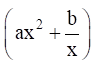Solution: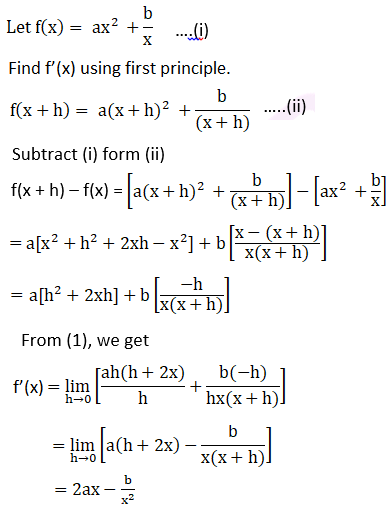Question 3: Derivate 3x2 + 2x – 5

Solution:

Let f(x) = 3x2 + 2x – 5….(i)

Find f’(x) using first principle.

Now,

f(x + h) = 3(x+h)2 + 2(x+h) – 5

= 3x2 + 3h2 + 6xh + 2x + 2h – 5 …..(ii)

Subtract (i) form (ii)

f(x + h) – f(x) = 3x2 + 3h2 + 6xh + 2x + 2h – 5 – 3x2 – 2x + 5

= 3h2 + 6xh + 2h

From (1), we get

f’(x) = lim(h->0) {(3h2 + 6xh + 2h)/h} = 6x + 2

Question 4: Derivate x3 – 2x2 + x + 3

Solution:

Let f(x) = x3 – 2x2 + x + 3 …….(i)

Find f’(x) using first principle.

Now,

f(x + h) = (x+h)3 – 2(x+h)2 + (x + h) + 3 …..(ii)

Subtract (i) form (ii)

f(x + h) – f(x) = (x+h)3 – 2(x+h)2 + (x + h) + 3 – x3 + 2x2 – x – 3

= [(x+h)3 – x3] – 2[(x+h)2 – x2] + [x + h – x] [Using the identities:

(a + b) 3 = a3 + b3 + 3ab2 + 3a2b

(a + b) 2 = a2 + b2 + 2ab ]

= h3 + 3xh2 + 3x2h – 2[h2 + 2xh] + h

From (1), we get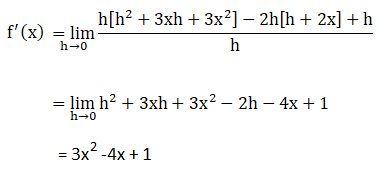Question 5: Derivate x8

Solution:

Let f(x) = x8

Find f’(x) using first principle.

Now,

f(x + h) = (x+h)8

From (1), we get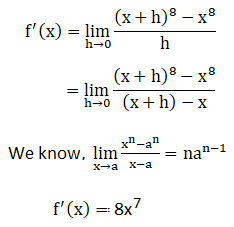Question 6: Derivate 1/x3

Solution:

Let f(x) = 1/x3

Find f’(x) using first principle.

Now,

f(x + h) = 1/(x+h)3

From (1), we get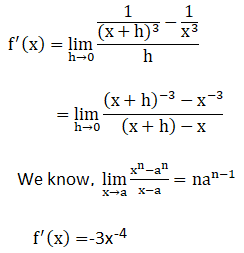Question 7: Derivate 1/x5

Solution:

Let f(x) = 1/x5

Find f’(x) using first principle.

Now,

f(x + h) = 1/(x+h)5

From (1), we get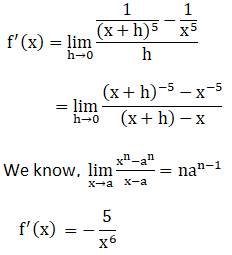Question 8: Derivate √(ax+b)

Solution:

Let f(x) = √(ax+b)

Find f’(x) using first principle.

Now,

f(x + h) = √(a(x+h) +b)

From (1), we get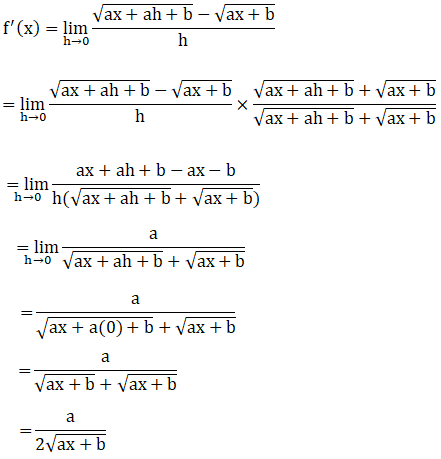Question 9: Derivate √(5x-4)

Solution:

Let f(x) = √(5x-4)

Find f’(x) using first principle.

Now,

f(x + h) = √(5(x+h) – 4)

From (1), we get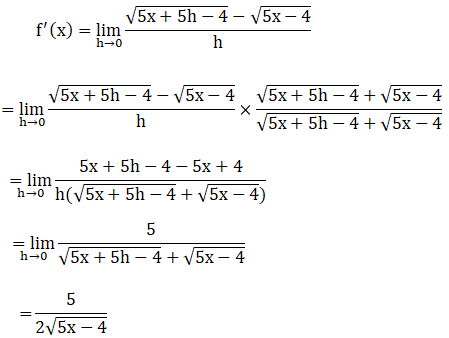Question 10: Derivate 1/√(x+2)

Solution:

Let f(x) = 1/√(x+2)

Find f’(x) using first principle.

Now,

f(x + h) = 1/√((x+h) +2)

From (1), we get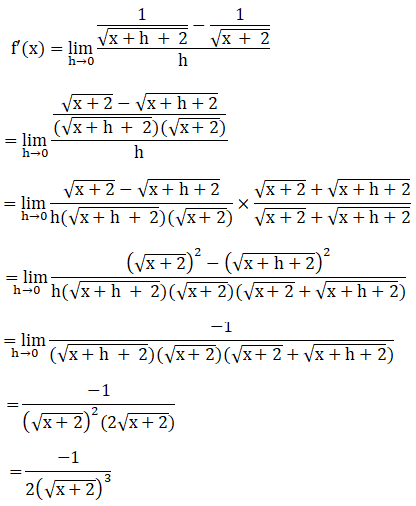Question 11: Derivate 1/√(2x+3)

Solution:

Let f(x) = 1/√(2x+3)

Find f’(x) using first principle.

Now,

f(x + h) = 1/√(2(x+h) +3)

From (1), we get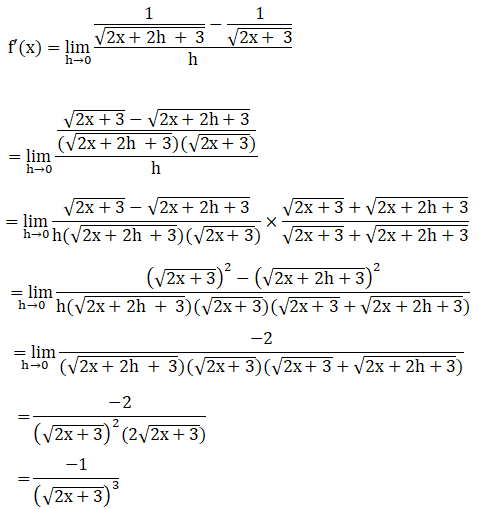Question 12: Derivate 1/√(6x-5)

Solution:

Let f(x) = 1/√(6x-5)

Find f’(x) using first principle.

Now,

f(x + h) = 1/√(6(x+h) – 5)

From (1), we get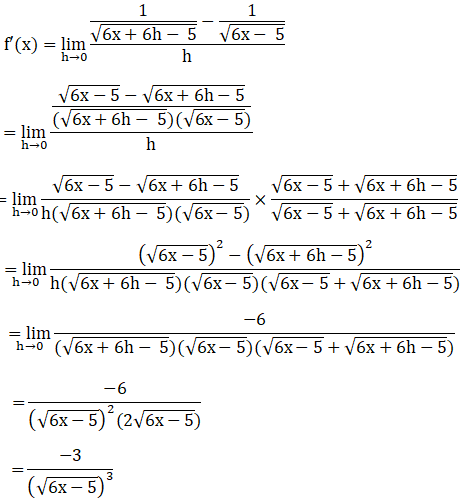Exercise 28C Page No: 882

Question 1: Differentiate: x2 sin x

Solution:

By Product Rule: (uv)′ = u′v + uv′

Here u = x2 and v = sin x

(x2 sin x)’ = 2x (sinx) + x2 (cosx)

= 2xsinx + x2cosx

Question 2: Differentiate ex cos x

Solution:

By Product Rule: (uv)′ = u′v + uv′

Here u = ex and v = cos x

(ex cosx)’ = ex (cosx) + ex (-sinx)

= excosx – exsinx

= ex (cosx – sinx)

Question 3: Differentiate ex cot x

Solution:

By Product Rule: (uv)′ = u′v + uv′

Here u = ex and v = cot x

(ex cotx)’ = ex (cotx) + ex ( -cosec2x)

= ex (cotx) – ex cosec2x

= ex (cotx – cosec2x)

Question 4: Differentiate xn cot x

Solution:

By Product Rule: (uv)′ = u′v + uv′

Here u = xn and v = cot x

(xn cotx)’ = nxn-1 (cotx) + xn (-cosec2x)

= nxn-1 (cotx) – xn (cosec2x)

= xn-1 (n cotx – x cosec2x)

Question 5: Differentiate x3 sec x

Solution:

By Product Rule: (uv)′ = u′v + uv′

Here u = x3 and v = sec x

(x3 sec x)’ = 3x2 (secx) + x3 (secx tanx)

= x2secx(3 + x tanx)

Question 6: Differentiate (x2 + 3x + 1) sin x

Solution:

By Product Rule: (uv)′ = u′v + uv′

Here u = (x2 + 3x + 1) and v = sin x

[(x2 + 3x + 1) sin x]’ = (2x + 3) × sinx + (x2 + 3x + 1) × cosx

= (2x + 3) sinx + (x2 + 3x + 1) cosx

Question 7: Differentiate x4 tan x

Solution:

By Product Rule: (uv)′ = u′v + uv′

Here u = x4 and v = tan x

(x4 tan x)’ = 4x3 × tanx + x4 × sec2x

= 4x3tanx + x4sec2x

Exercise 28D Page No: 886

Solve all the questions using quotient rule.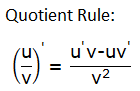………(1)

Question 1: Differentiate 2x/x

Solution:

Here u = 2x and v = x

u’ = d/dx (2x) = 2x log 2

v’ = d/dx (x) = 1

(1)=>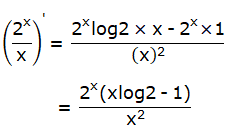Question 2: Differentiate log x/x

Solution:

Here u = log x and v = x

u’ = d/dx (log x) = 1/x

v’ = d/dx (x) = 1

(1)=>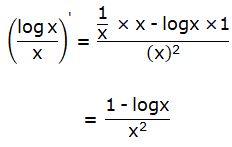Question 3: Differentiate ex/(1+x)

Solution:

Here u = ex and v = 1+x

u’ = d/dx (ex) = ex

v’ = d/dx (1+x) = 1

(1)=>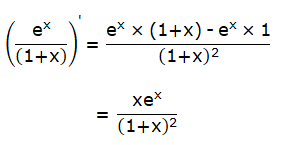Question 4: Differentiate ex/(1+x2)

Solution:

Here u = ex and v = 1+x2

Using Quotient rule, we have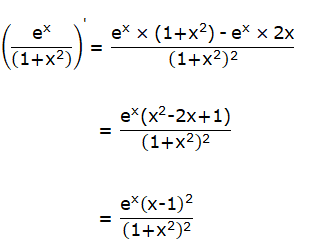Question 5: Differentiate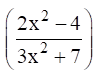Solution:

Here u = 2x2 – 4 and v = 3x2 + 7

Using Quotient rule, we have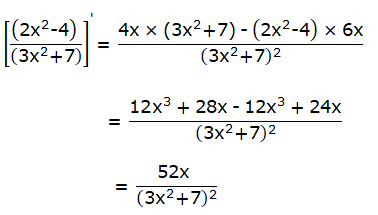Question 6: Differentiate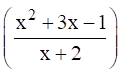Solution:

Here u = x2 +3x – 1 and v = x + 2

Using Quotient rule, we have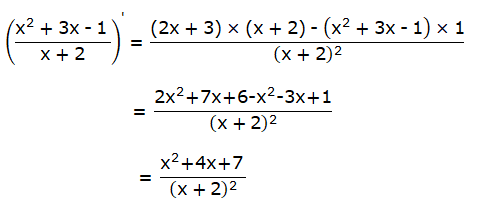Question 7: Differentiate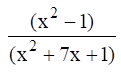Solution:

Here u = x2 – 1 and v = x2 + 7x + 1

Using Quotient rule, we have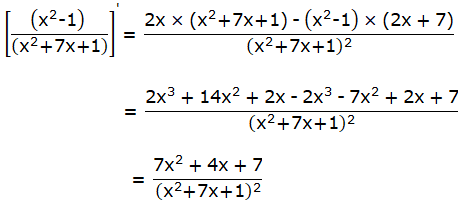Question 8: Differentiate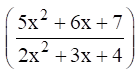Solution:

Here u = 5x2 +6x + 7 and v = 2x2 + 3x + 4

Using Quotient rule, we haveQuestion 9: Differentiate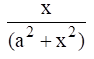Solution:

Here u = x and v = a2 + x2

Using Quotient rule, we have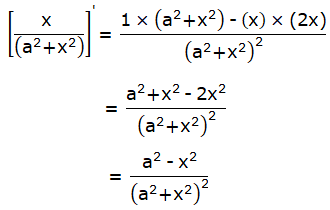Question 10: Differentiate x4/sinx

Solution:

Here u = x4 and v = sinx

Using Quotient rule, we have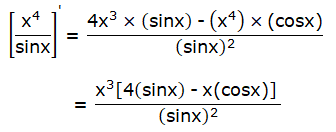Question 11: Differentiate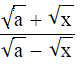Solution:

Here u = √a + √x and v = √a – √x

Using Quotient rule, we have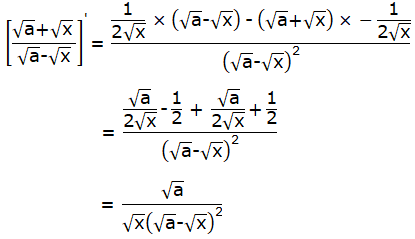Question 12: Differentiate cosx/log x

Solution:

Here u = cos x and v = log x

Using Quotient rule, we have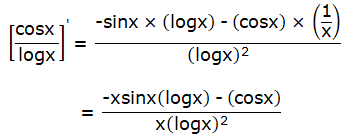Exercise 28E Page No: 892

Differentiate the following with respect to x:

Differentiate below functions using “Chain Rule”:

If y = f(t) and t = g(x) then dy/dx = dy/dt x dt/dx

Question 1: Differentiate sin 4x

Solution:

Let y = sin 4x

If 4x = t then sin4x = sin t or y = sin t

dy/dt = cos t

dt/dx = 4

Now,

dy/dx = dy/dt x dt/dx = 4 cos t

Substituting the value of t back, we get

dy/dx = 4 cos 4x

Question 2: Differentiate cos 5x

Solution:

Let y = cos 5x

If t = 5x then cos 5x = cos t or y = cos t

dy/dt = -sin t

dt/dx = 5

Now,

dy/dx = dy/dt x dt/dx = -5 sin t

Substituting the value of t back, we get

dy/dx = -5 sin 5x

Question 3: Differentiate tan 3x

Solution:

Let y = tan 3x

If t = 3x then tan 3x = tan t or y = tan t

dy/dt = sec2 t

dt/dx = 3

Now,

dy/dx = dy/dt x dt/dx = 3 sec2 t

Substituting the value of t back, we get

dy/dx = 3sec2 3x

Question 4: Differentiate cos x3

Solution:

Let y = cos x3

If t = x3 then cos x3= cos t or y = cos t

dy/dt = -sin t

dt/dx = 3x2

Now,

dy/dx = dy/dt x dt/dx = 3 x2 (-sin t)

Substituting the value of t back, we get

dy/dx = 3 x2 (-sin x3)

= -3 x2 sin x3

Question 5: Differentiate cot2 x

Solution:

Let y = cot2 x

dy/dx = – 2cotx [d/dx (cot x)]

= 2 cot x cosec2x

Question 6: Differentiate tan3 x

Solution:

Let y = tan3 x

dy/dx = 3 tan2 x [d/dx (tan x)]

= 3 tan2 x sec2x

Question 7: Differentiate tan √x

Solution:

Let y = tan √x

dy/dx = sec2√x d/dx (√x)

= sec2√x {1/(2√x) }

= sec2√x / 2√x

Question 8: Differentiate ex^4

Solution: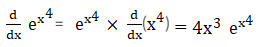Question 9: Differentiate ecotx

Solution:Question 10: Differentiate √(sin x)

Solution:

d/dx √(sin x) = d/dx (sin x)1/2

= 1/2 * (sinx)-1/2 * d/dx (sin x)

= 1/2 * (sinx)-1/2 * cos x

= cosx / 2√(sin x)

Question 11: Differentiate (5 + 7x)6

Solution:

d/dx(5 + 7x)6 = 6(5 + 7x)5 * d/dx(5 + 7x)

= 6(5 + 7x)5 * 7

= 42(5 + 7x)5

Question 12: Differentiate (3 – 4x)5

Solution:

d/dx(3 – 4x)5 = 5(3 – 4x)4 * d/dx (3 – 4x)

= 5(3 – 4x)4 * ( – 4)

= – 20(3 – 4x)4

Question 13: Differentiate (3x2 – x + 1)4

Solution:

d/dx (3x2 – x + 1)4 = 4(3x2 – x + 1)3 * d/dx (3x2 – x + 1)

= 4(3x2 – x + 1)3 * (6x – 1)

= 4(3x2 – x + 1)3 (6x – 1)

Question 14: Differentiate (ax2 + bx + c)n

Solution:

d/dx (ax2 + bx + c)n = n(ax2 + bx + c)n-1 * d/dx (ax2 + bx + c)

= n(ax2 + bx + c)n-1 (2ax + b)

Question 15: Differentiate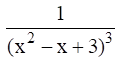Solution:

d/dx (x2 – x + 3)-3 = -3(x2 – x + 3)-4 * d/dx (x2 – x + 3)

= -3(x2 – x + 3)-4(2x2 – 1)

Question 16: Differentiate sin2 (2x + 3)

Solution:

d/dx sin2 (2x + 3) = 2 sin (2x + 3) * d/dx {sin(2x + 3)}

= 2 sin (2x + 3) * cos(2x + 3) * d/dx (2x + 3)

= 2 sin (2x + 3) * cos(2x + 3) * 2

= 4 sin(2x + 3) cos(2x + 3)

= 2 sin (4x + 6)

[Using identity: 2sin x cos x = sin 2x]

Question 17: Differentiate cos2(x3)

Solution:

d/dx cos2(x3) = 2 cos(x3) * d/dx { cos (x3)}

= 2 cos(x3) * ( – sin (x3)) * d/dx (x3)

= 2 cos(x3) * ( – sin (x3)) * 3x2

= – 6x2 cos(x3) sin x3

Question 18: Differentiate √(sin (x3 ))

Solution: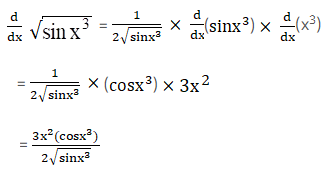Question 19: Differentiate √(x sin x)

Solution: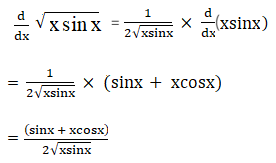Question 20: Differentiate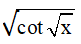Solution: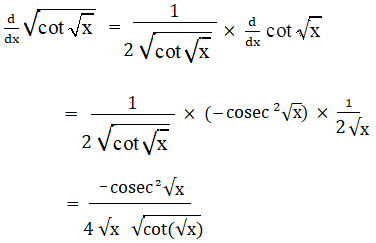## R S Aggarwal Solutions For Class 11 Maths Chapter 28 Exercises:

Get detailed solutions for all the questions listed under below exercises:

Exercise 28A Solutions

Exercise 28B Solutions

Exercise 28C Solutions

Exercise 28D Solutions

Exercise 28E Solutions

## R S Aggarwal Solutions for Class 11 Maths Chapter 28 Differentiation

R S Aggarwal Chapter 28 – Differentiation, contains the answers to all the questions listed in this chapter. Let us have a look at some of the topics covered here:

• Differentiation Introduction
• Derivative of a function
• Differentiation from first principle
• Derivative of the product of functions
• Derivative of the quotient of two functions